• 基础知识
• 流水线

# 第一章 基础知识

• 计算机系统层次结构
• 计算机系统结构基本概念（广义机器、透明性、编译）
• 计算机系统结构、组织、实现的定义
• 计算机系统分类方法/Flynn 分类法

• 大概率事件优先
• Amdahl 定律
• 程序的局部性原理
• CPU 性能计算
• 加速比公式应用

• 性能指标（CPU 时间、CPI、MIPS、MFLOPS）

## 计算机系统结构相关概念

0 布尔语言（硬件）
1 微程序指令 用微指令集编写微程序，固件、硬件来解释
2 传统机器语言 传统机器语言程序有 L1 级微程序或 L0 级硬联逻辑进行解释
3 操作系统 包括传统机器及操作系统级指令，由微程序解释
4 汇编语言 翻译成 L3 和 L2 级语言执行
5 高级语言 通过编译程序翻译到 L4 或 L3 级，或通过解释方法实现
6 应用语言 由应用程序包翻译到 L5

• 翻译：用转换程序把高一级机器上的程序转换为低一级机器上的等效程序，然后再在这低一级机器上运行。速度快、占用空间大。
• 解释：对于高一级机器上程序中的每一条语句或指令，转换为低级语言的一段等效程序执行，执行完后再去高一级机器取下一条语句或指令。速度慢、占用空间小。

• 计算机系统结构是指传统机器程序员所看到的计算机属性，即概念性结构功能特性
• 计算机系统结构的实质：确定计算机系统中软硬件的界面，界面之上是软件实现的功能，界面之下是硬件和固件实现的功能。
• 透明性：一种本来存在的事物或属性，从某种角度看好像不存在或看不到。低层机器的属性对高层机器程序员来说通常是透明的。
• 广义系统结构定义：包括指令系统结构、组成、硬件。

• 计算机系统结构：数据表示、寻址规则、寄存器定义、指令集、终端系统、机器工作状态的定义和切换、存储体系、信息保护、I/O 结构等。

• 计算机组成：计算机系统结构的逻辑实现，包含物理机器级中的数据流和控制流的组成以及逻辑设计等。着眼于：物理机器级内各事件的排序方式与控制方式、各部件的功能以及各部件之间的联系。具有相同系统结构的计算机可以采用不同的计算机组成。

• 计算机实现：计算机组成的物理实现。着眼于：器件技术、微组装技术。同一种计算机组成又可以采用多种不同的计算机实现。

• 机器指令集的确定、主存容量与编址方式等属于计算机体系结构。
• 指令实现方式（取指令、去操作数、运算、送结果等的具体操作及排序方式）、主存速度与逻辑结构（多体交叉存储）等属于计算机组织（计算机组成）。
• 实现指令集中所有指令功能的具体电路、器件的设计、装配技术，存储器器件和逻辑电路的设计等属于计算机实现。

• Flynn 分类法：按照指令流和数据流的多倍性分类。

• 指令流：计算机执行的指令序列。
• 数据流：由指令流调用的数据序列。
• 多倍性：在系统最受限的部件上，同时处于统一执行阶段的指令或数据的最大数目。

分为 SISD（传统顺序处理计算机）、SIMD（阵列处理机）、MISD（流水处理机，有争议）、MIMD（多处理机）系统。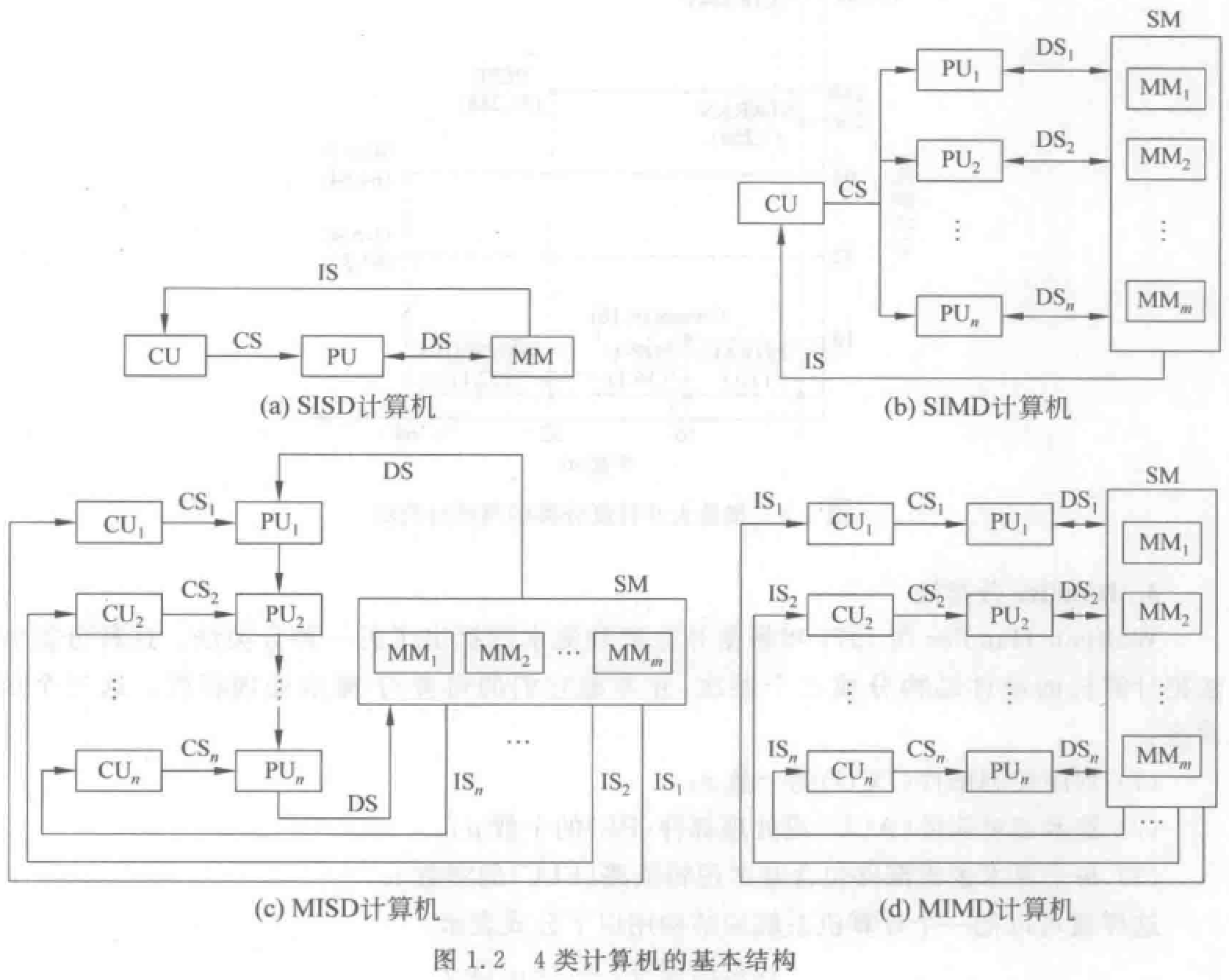• 冯氏分类法：用最大并行度$P_m$（单位时间内能够处理的最大二进制位数）分类。

• 字串位串 WSBS：纯串行处理机
• 字串位并 WSBP：传统单处理机
• 字并位串 WPBS：同时处理多个字的同一位
• 字并位并 WPBP：同时处理多个字的多个位

平均并行度：假设每个时钟周期内能同时处理的二进制位数为$P_i$，则$T$个时钟周期内的平均并行度为$p_a = \frac{\sum_{i=1}^T P_i}{T}$$T$个周期内的平均利用率：$\mu = \frac{P_a}{P_m}$

• Handler 分类法：把硬件结构分为三个层次，根据并行度和流水线分类。

$T=<k\times k', d \times d', w \times w'>$

$k$：控制器数目，$k'$：控制器流水线中控制部件的数目

$d$：PCU 控制的 ALU 或 PE 数目，$d'$：指令流水线中 ALU 部件的数目

$w$：ALU 或 PE 的字长，$w'$：操作流水线中基本逻辑线路数目

例题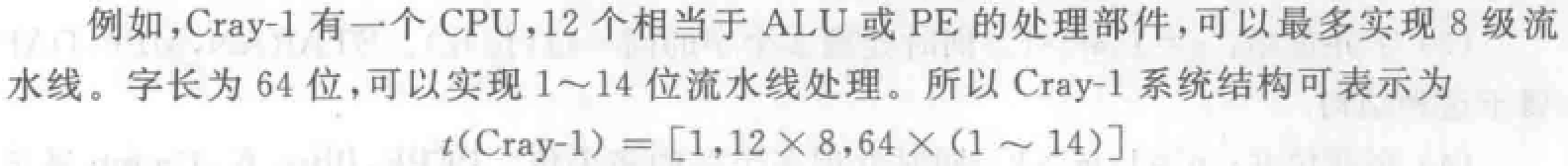• 冯诺依曼结构：输入设备、输出设备、控制器、运算器、存储器。指令与数据同等对待（一条总线）。
• 哈佛结构：冯诺依曼基础上，指令与数据分离。现代计算机都是哈佛结构。

• “由上往下”设计。
• “由下往上”设计。
• “由中间开始”设计：从传统机器级与操作系统机器级开始。

• 同时性：两个或两个以上的事件在同一时刻发生。
• 并发性：两个或两个以上的事件在同一时间间隔内发生。

• 字串位串：每次只对一个字的一位进行处理。
• 字串位并：同时对一个字的全部位进行处理，不同字之间是串行的。
• 字并位串：同时对许多字的同一位（称为位片）进行处理。
• 全并行：同时对许多字的全部位或部分位进行处理。

• 指令内部并行：单条指令中各微操作之间的并行。
• 指令级并行。
• 线程级并行。
• 任务级或过程级并行：以子程序或进程为调度单元。
• 作业或程序级并行。

• 时间重叠：轮流使用同一套硬件的各个部分。
• 资源重复：重复设置硬件资源。
• 资源共享：按顺序轮流使用同一套硬件设备。

## 基本原理和性能公式

• 时间局部性：程序即将用到的信息很可能就是目前正在使用的信息。（经验规则：程序执行时间 90%都是在执行程序中 10%的代码）
• 空间局部性：程序即将用到的信息很可能与正在使用的信息在空间上临近。

Amdahl 定律：系统性能加速比与该部件在系统中的总执行时间有关。

• $加速比 S_n=\frac{改进后性能}{改进前性能}=\frac{改进前用时}{改进后用时}$

• $S_n = \frac{T_0}{T_n} = \frac{1}{(1-F_e)+\frac{F_e}{S_e}}$$F_e$为可改进比例，$S_e$为部件加速比。

例题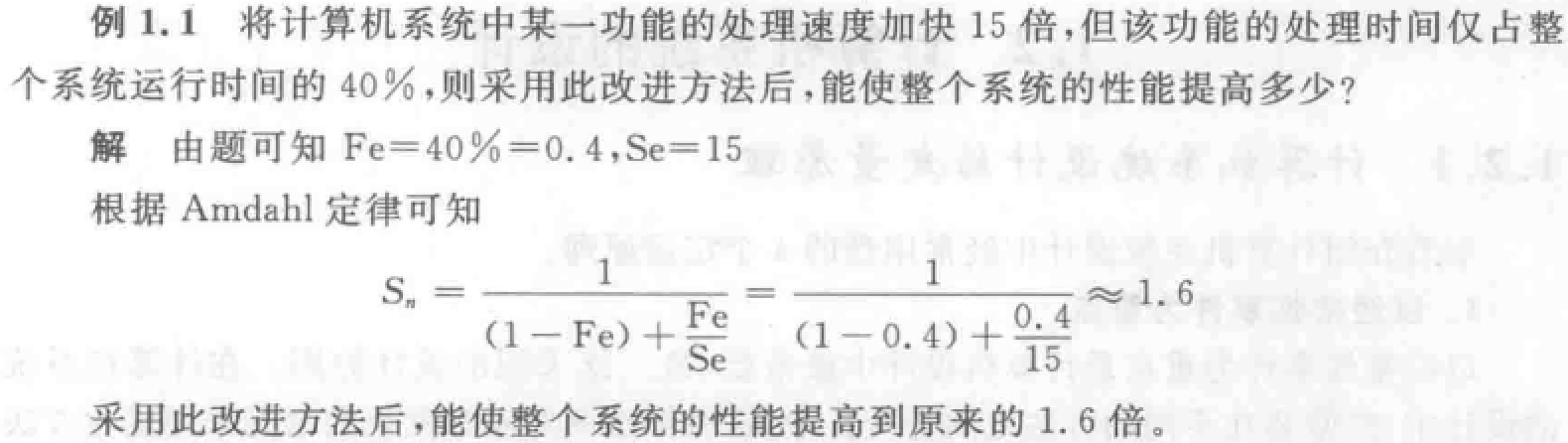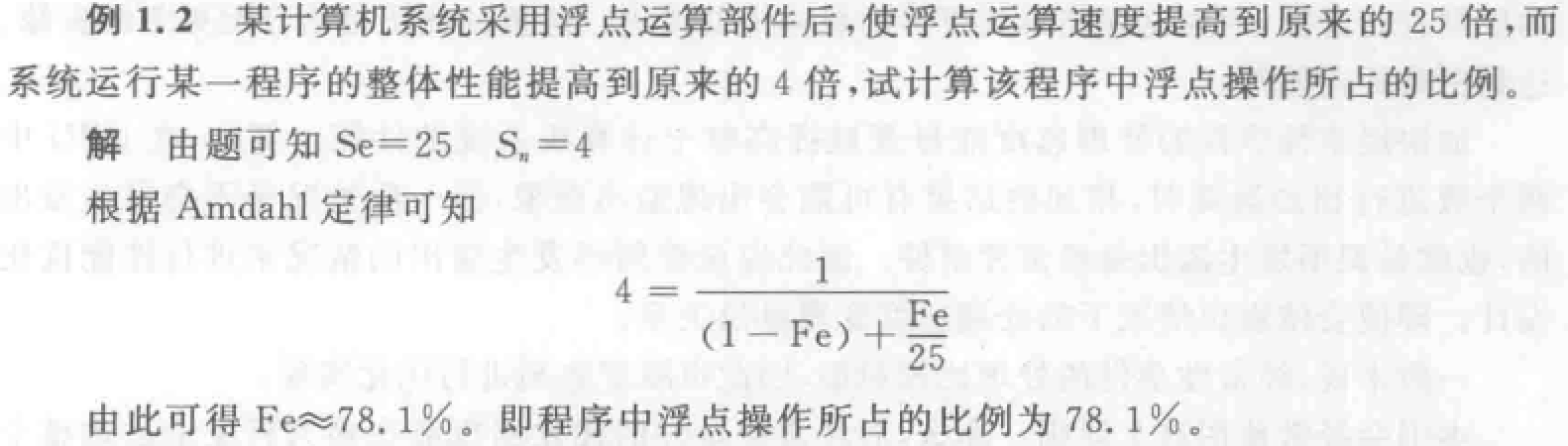CPU 性能公式：

• CPU 时间：执行一个程序所需 CPU 时间。

• $CPU 时间=时钟周期数 \times 时钟周期时间=IC \times CPI \times 时钟周期时间$。时钟周期时间$t = 1/f（系统时钟频率）$
• $时钟周期数=\sum\limits_{i=1}^n (CPI_i \times IC_i)$
• CPI：一条指令的平均时钟周期数，$=执行程序所需的时钟周期数 \div IC（执行程序的指令条数）$

• MIPS：每秒百万条指令数，$=f/CPI$

• MFLOPS：每秒百万次浮点运算数

• CPU 性能取决于三个参数：

• t：取决于硬件实现技术和计算机组成
• CPI：取决于计算机组成和指令系统的结构
• IC：取决于指令系统的结构和编译技术

例题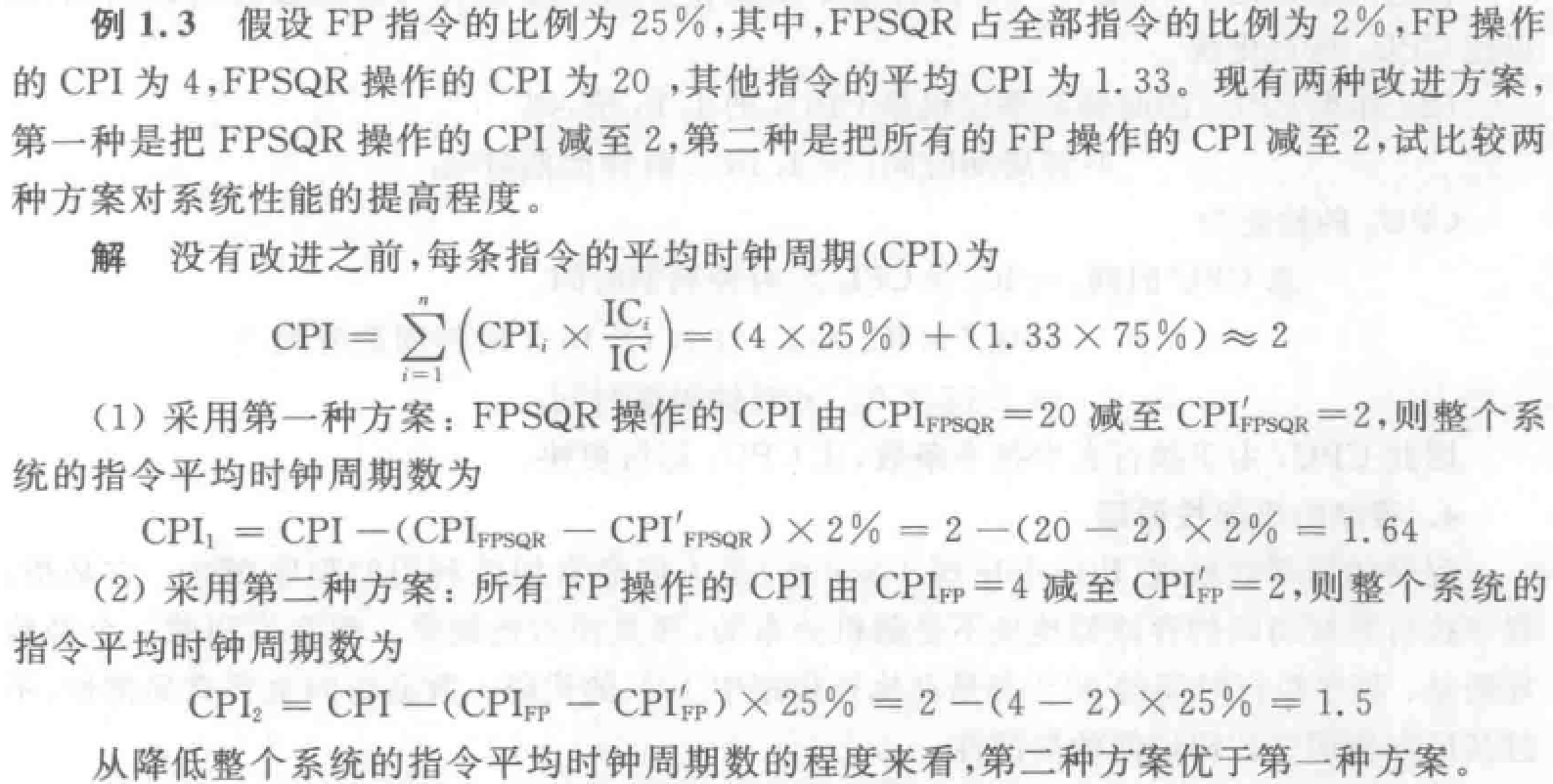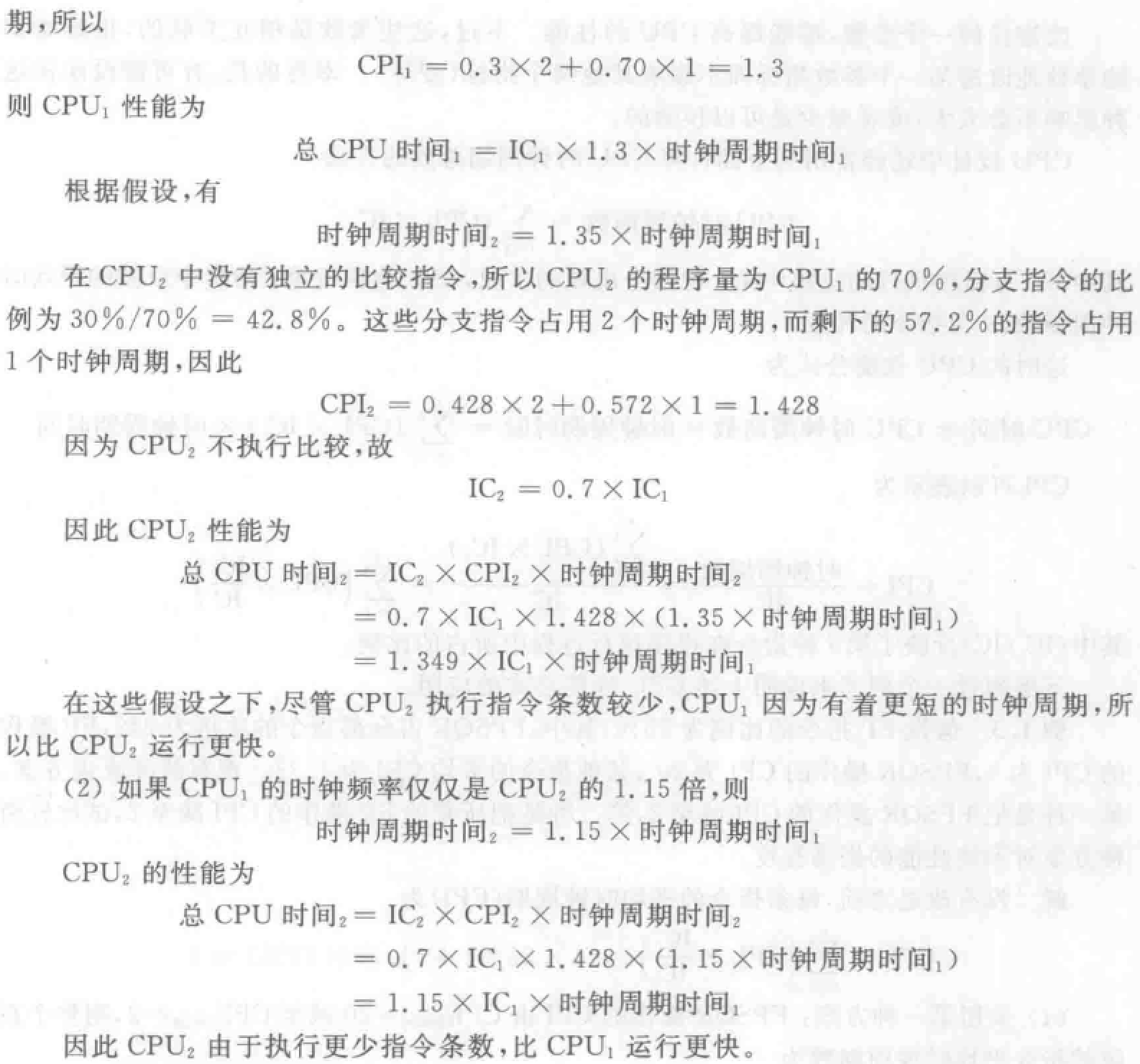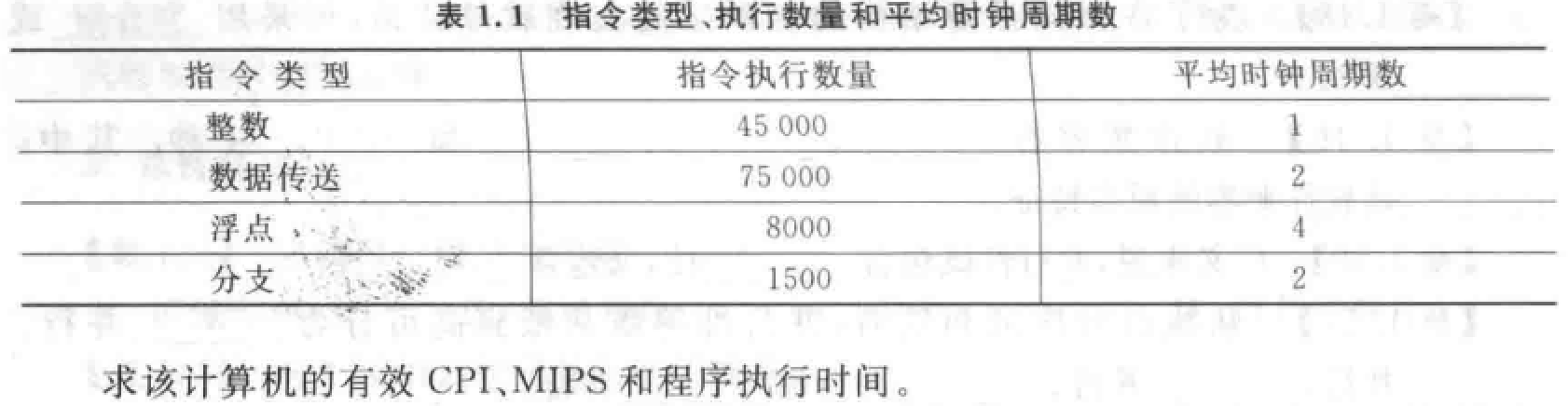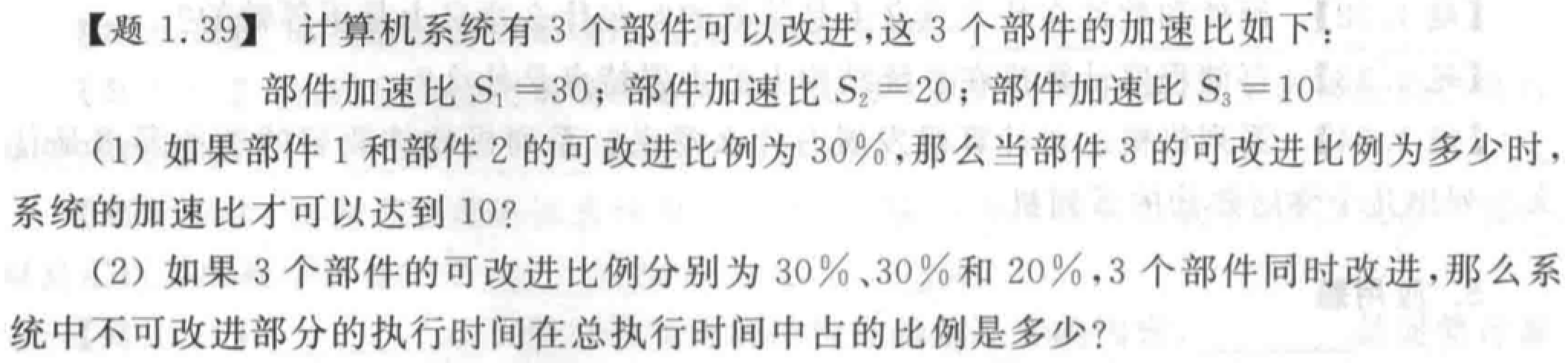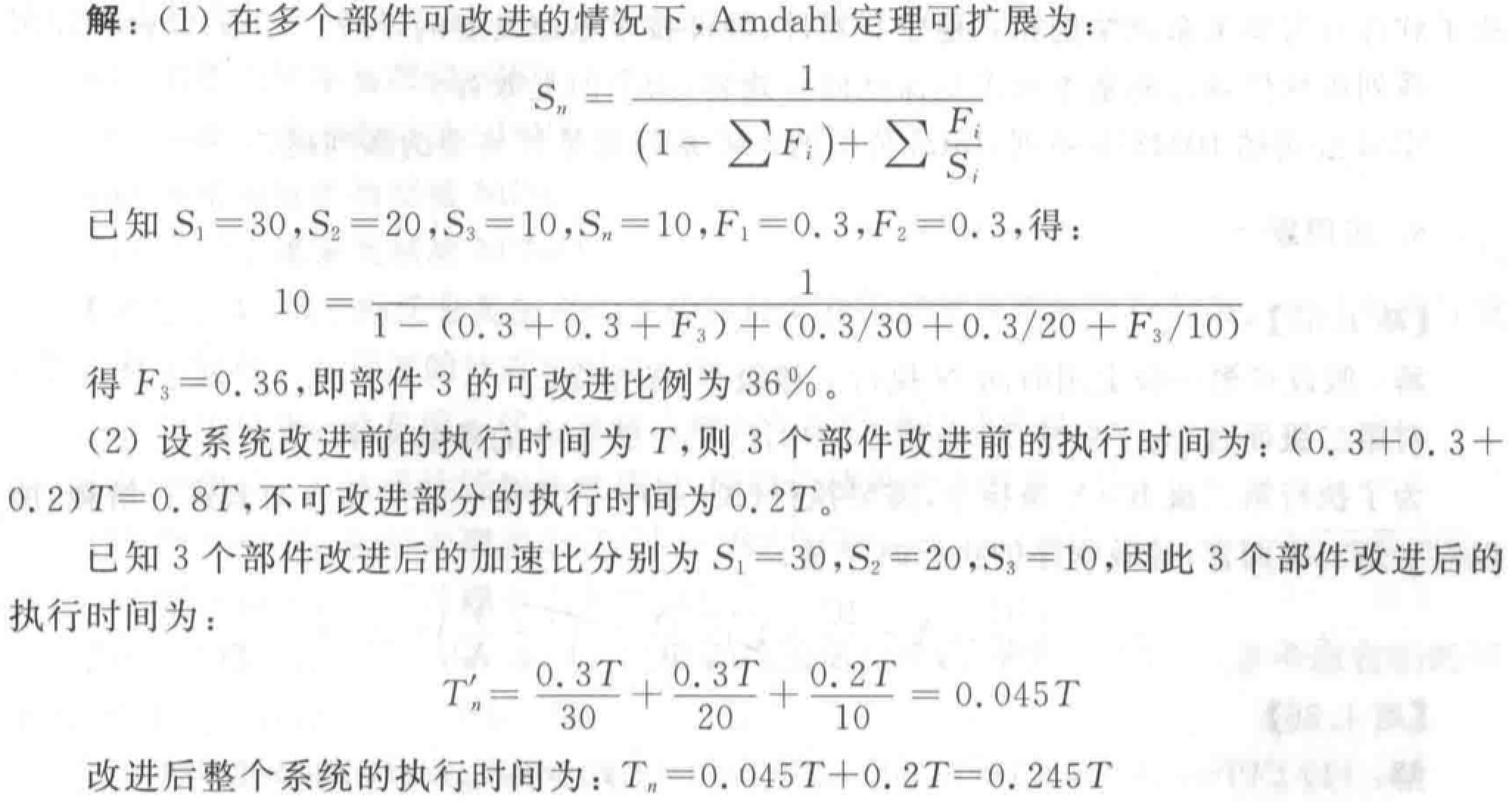## 性能评价标准

“X 的性能是 Y 的 n 倍”：$n=\frac{执行时间 Y}{执行时间 X}=\frac{性能 x}{性能 y}$

CPU 时间 = 用户 CPU 时间 + 系统 CPU 时间

• 平均执行时间：各程序执行时间的算术平均值。$S_m=\frac 1n \sum_{i=1}^n T_i$
• 加权执行时间：各程序执行时间的加权平均值。$A_m=\sum_{i=1}^n W_iT_i$
• 调和平均值法：执行任务数量$\div$总耗时（速度不可简单相加）。$H_m = \frac{n}{\sum_{i=1}^n \frac 1{R_i}}=\frac{n}{\sum_{i=1}^n T_i}$
• 几何平均值法：执行速度的几何均值。$G_m = \sqrt[n]{\prod_{i=1}^n R_i} =\sqrt[n]{\prod_{i=1}^n \frac 1{T_i}}$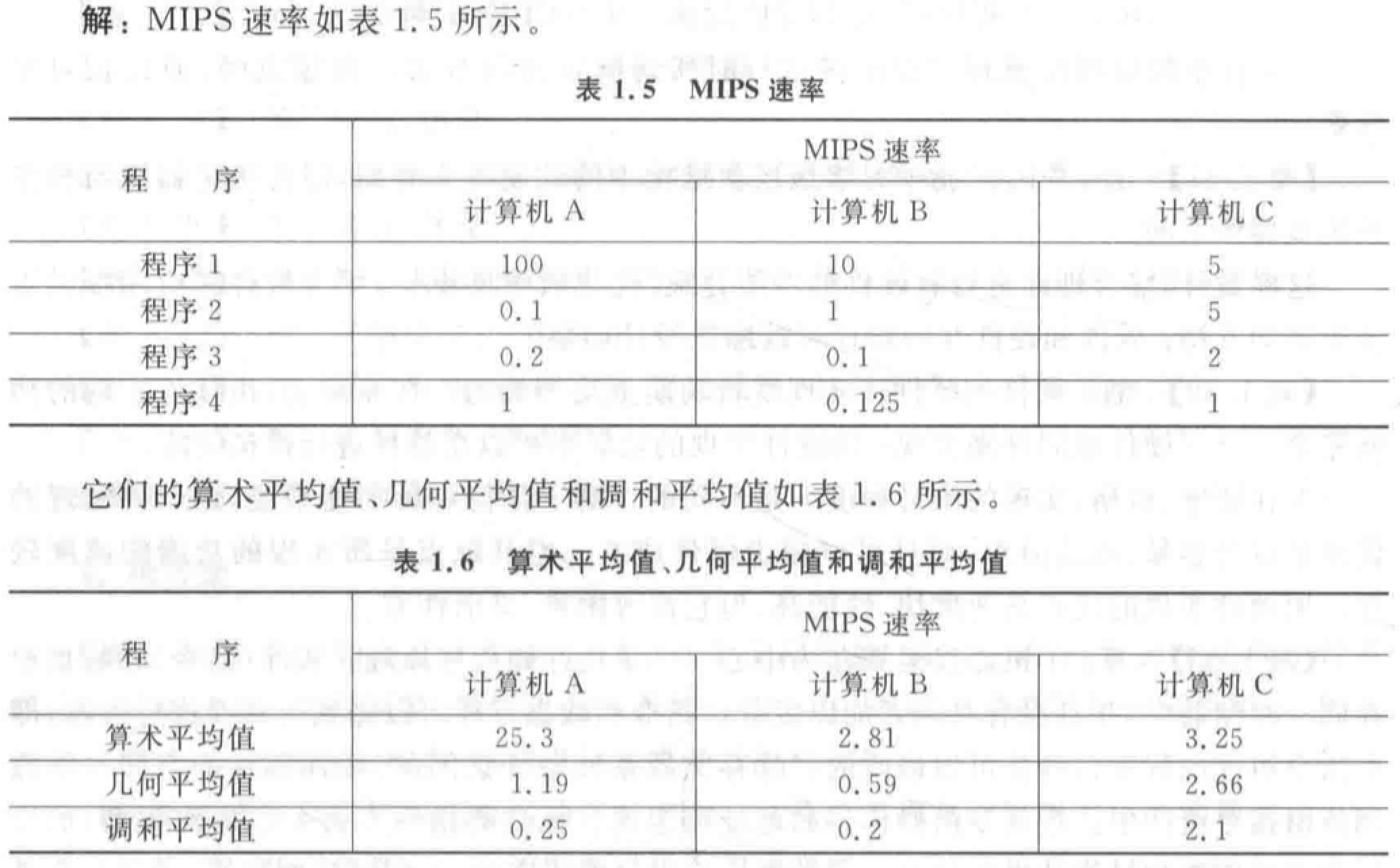# 第三章 流水线技术

• 经典五段流水线：各段完成的操作

• 数据相关（真相关）、名相关、控制相关
• 结构冲突、数据冲突、控制冲突
• 数据冲突的各种形式（RAW、WAR、WAW 等）
• 减少数据冲突的方法：延迟、定向、编译

• 解决控制冲突的方法：排空、预测、延迟分支、编译

## 流水线基本概念及分类

• 操作部件采用流水线，称为操作部件流水线。
• 指令执行过程采用流水线，称为指令流水线。
• 访问主存部件采用流水线，称为访存部件流水线。
• 多计算机通过存储器连接构成流水线，称为宏流水线。

• 部件级流水线（运算操作流水线）：各类型的运算操作按流水方式进行。
• 处理机级流水线（指令流水线）：把一条指令的执行过程分段，按流水方式执行。
• 系统级流水线（宏流水线）：把多台处理机串行连接起来，每个处理机完成整个任务中的一部分。

• 单功能流水线：只能完成固定功能。
• 多功能流水线：流水线的各段可以进行不同连接，实现不同功能。

• 静态流水线：同一时间内，多功能流水线中的各段只能按同一种功能的连接方式进行工作。
• 动态流水线：同一时间内，多功能流水线中的各段可以按照不同方式连接，同时执行多种功能。（而不需等到排空之后重新装入）

• 线性流水线：流水线各段串行连接，每个段最多流过一次。
• 非线性流水线：流水线中有反馈回路，每个段可以流过多次。

• 顺序流水线：流水线输出的任务顺序与输入的任务顺序相同。
• 乱序流水线：流水线输出的任务顺序与输入的顺序可以不同。（也称无序、错序、异步流水线）

• 三段：取指、分析、执行
• 四段：取指（访问主存取出指令并送到指令寄存器）、译码（形成操作数地址并读取操作数）、执行（完成指令的功能）、存结果（将运算结果写回寄存器或主存）

• 通过时间：第一个任务进入流水线流出结果所需的时间，又称装入时间

• 排空时间：最后一个任务从进入流水线到流出结果所需的时间

在装入和排空的过程中，流水线不满载。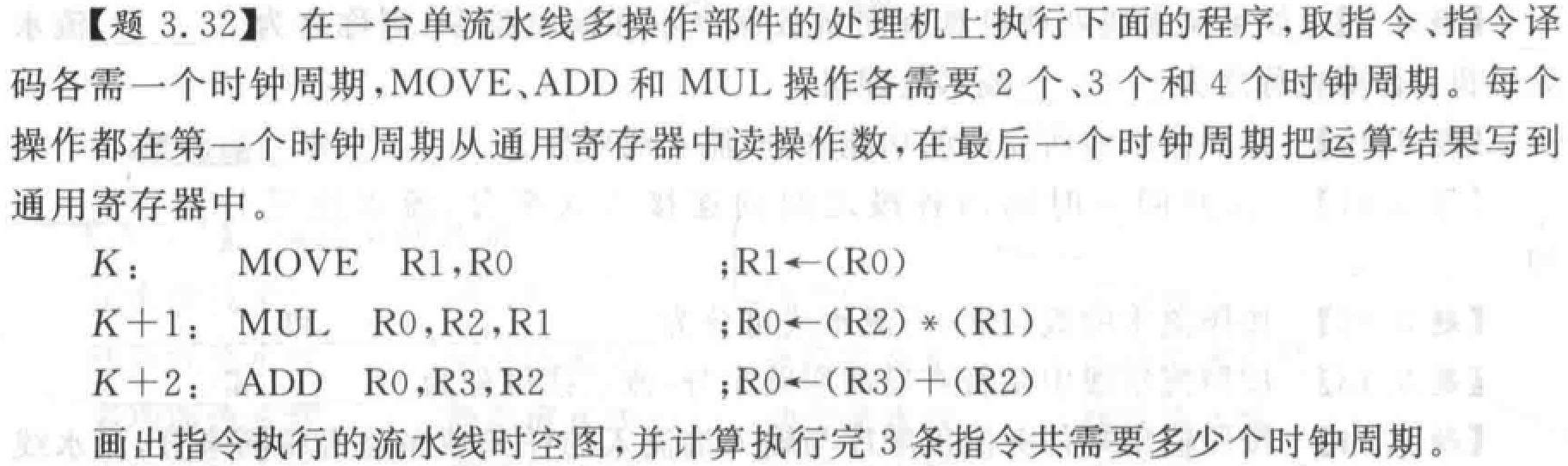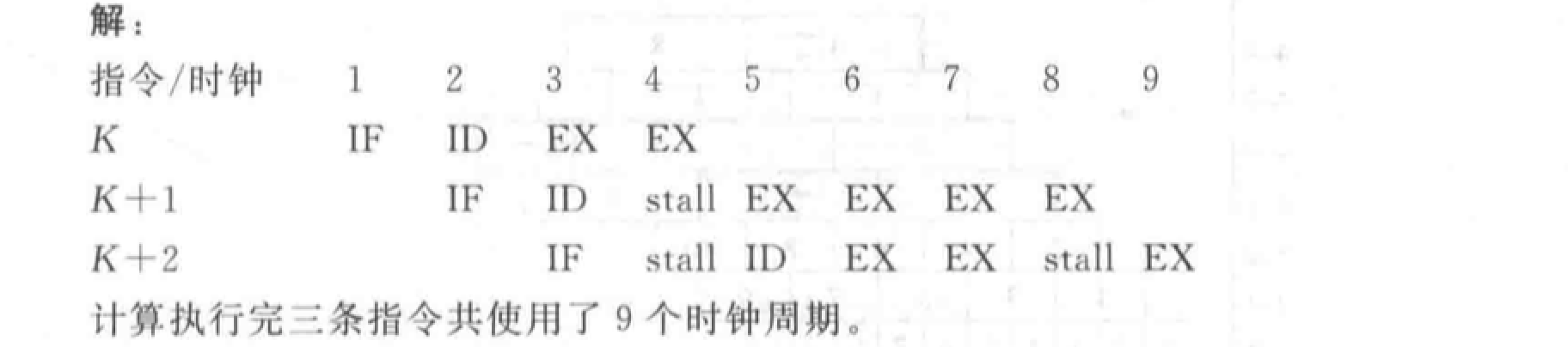4 周期 K+1 的 ID 停顿等待 K 写结果，K+2 的 IF 停顿等待释放 ID 部件。

9 周期 K+2 停顿等待 K+1 释放写回部件。

## 流水线性能计算和分析

• 各段时间相等的流水线：理想的 $k$ 段线性流水线完成 $n$ 个连续任务的时间：$T_k = (k+n-1) \Delta t$

实际吞吐率：$\frac{n}{(k+n-1) \Delta t}$

最大吞吐率：$TP_{max}=\lim\limits_{n \to \infty} \frac{n}{(k+n-1) \Delta t} = \frac{1}{\Delta t}$

• 各段时间不等的流水线：时间最长段称为流水线的瓶颈

实际吞吐率：$\frac{n}{\sum\limits_{i=1}^k \Delta t_i + (n-1) \max (\Delta t_1,\Delta t_2,···,\Delta t_k)}$

最大吞吐率：$TP_{max} = \frac{1}{\max (\Delta t_1,\Delta t_2,···,\Delta t_k)}$

• 各段时间相等的 $k$ 段流水线：$S = \frac{nk}{k+n-1}$

最大加速比：$k$

• 各段时间不等的流水线：$S = \frac{n \sum\limits_{i=1}^k \Delta t_i}{\sum\limits_{i=1}^k \Delta t_i + (n-1) \max (\Delta t_1,\Delta t_2,···,\Delta t_k)}$

• 连续完成 $n$ 个任务，每段的效率计算：每段都执行了 $n$ 个任务，用时 $n \Delta t$，效率 $e = \frac{n}{k+n-1}$
• 整条流水线的效率$E = \frac{e_1+e_2+...+e_k}{k}= \frac{n}{k+n-1}$

TP、S、E 关系

• $E=TP \times \Delta t$$E = \frac{S}{k}$

• 重复设置瓶颈段：让多个瓶颈流水段并行工作。
• 瓶颈段再细分：细分为多个子流水段（每个子流水段用时和非瓶颈段相同），形成超流水线。

• PCR 定义为单位价格的最大吞吐率。
• $PCR = \frac{P_{max}}{C}$，其中 $P_{max} = \frac{1}{\frac{t}{k}+d}$$C = \frac{1}{a+b \times k}$$t$ 为非流水机器串行完成一个任务的时间，$d$ 为锁存器延迟，$a$ 为流水段本身价格，$b$ 为锁存器价格。
• $k$ 求导可得 PCR 极大值，以及对应的最佳段数 $k_0= \sqrt{\frac{t \times a}{d \times b}}$
• 大于等于 8 段的流水线称为超流水线。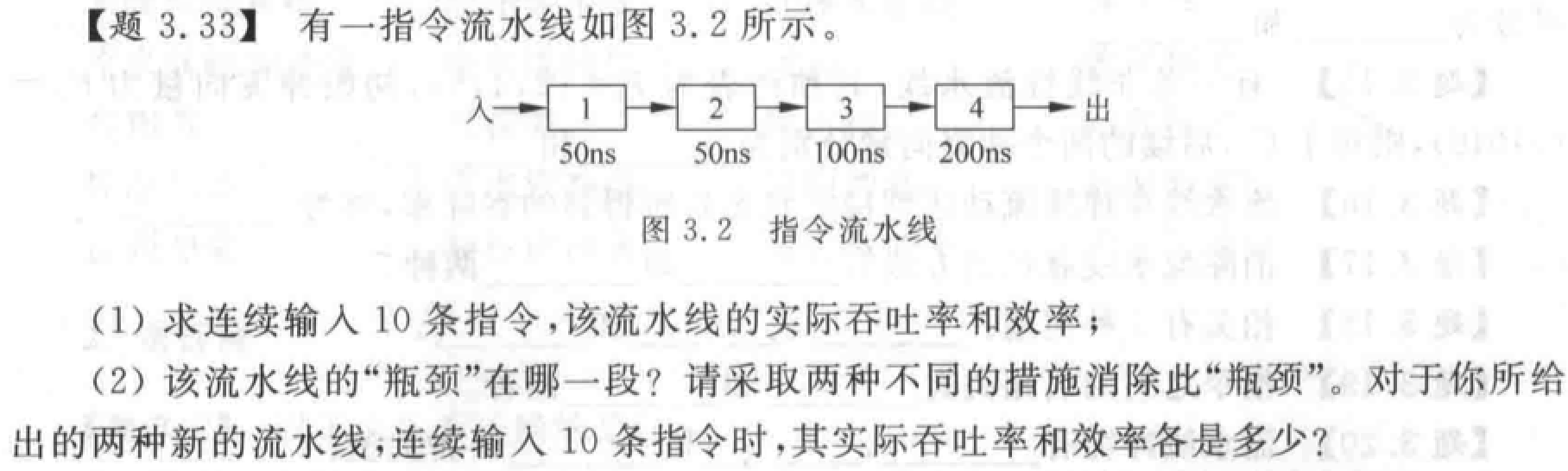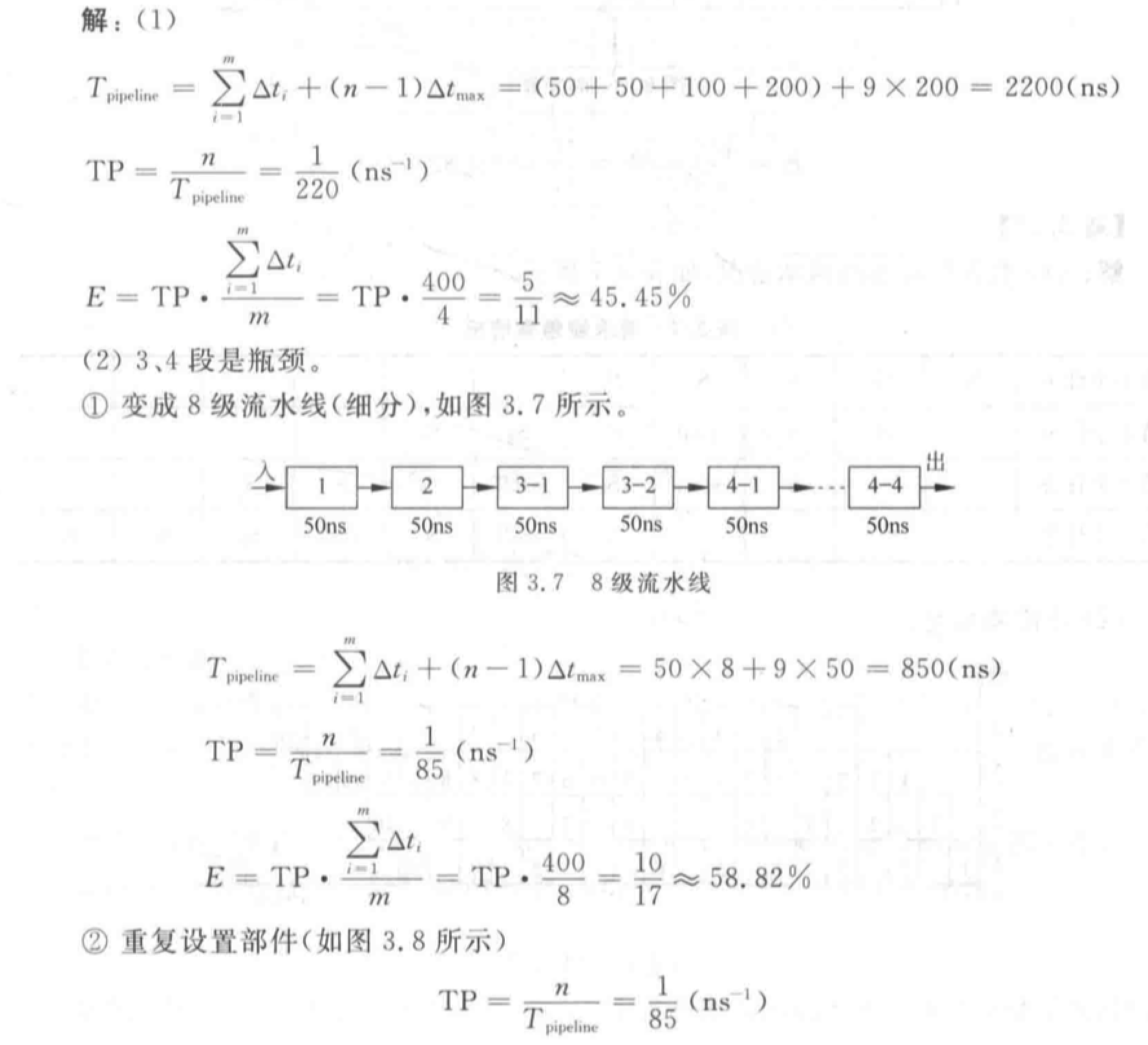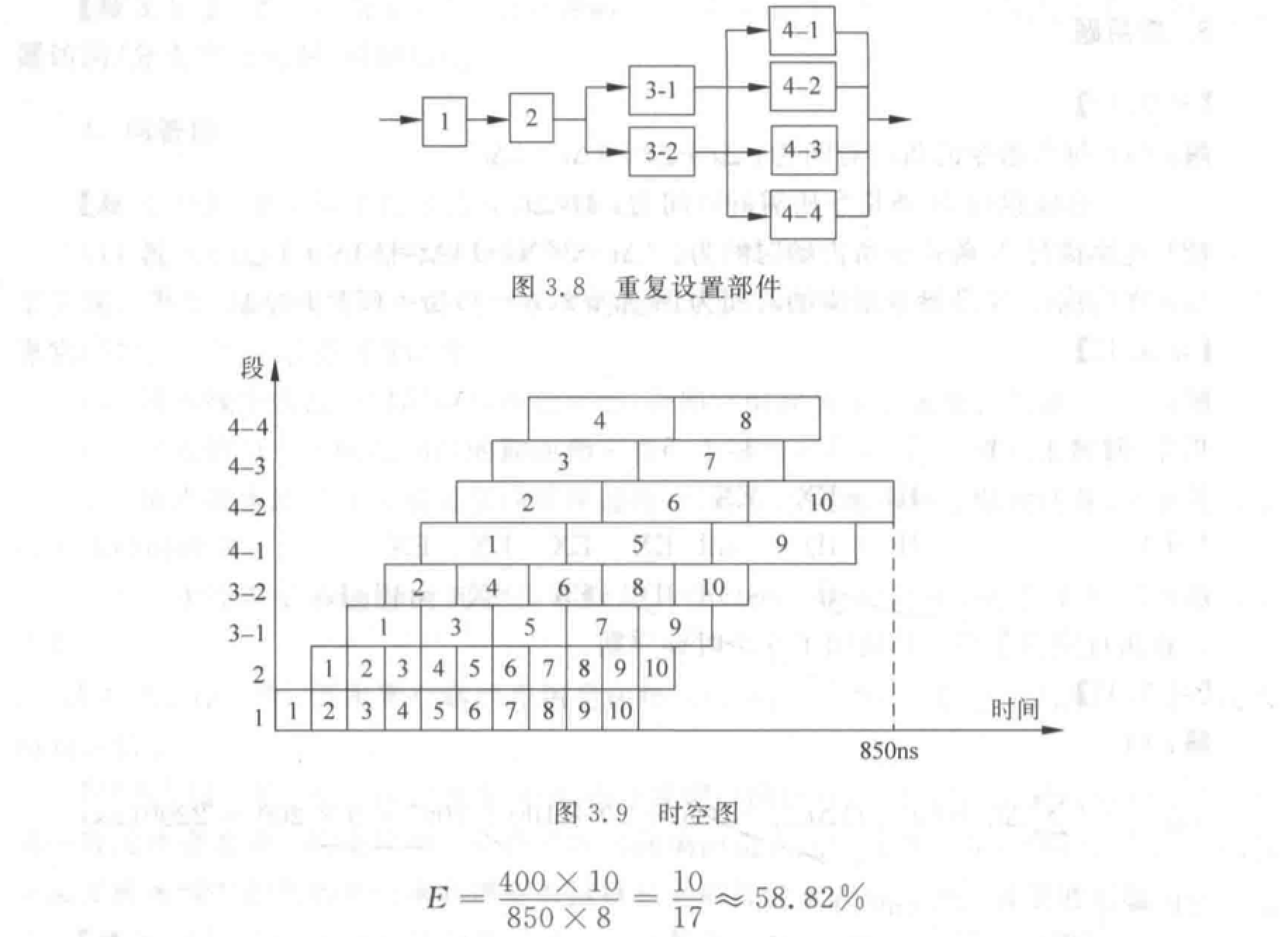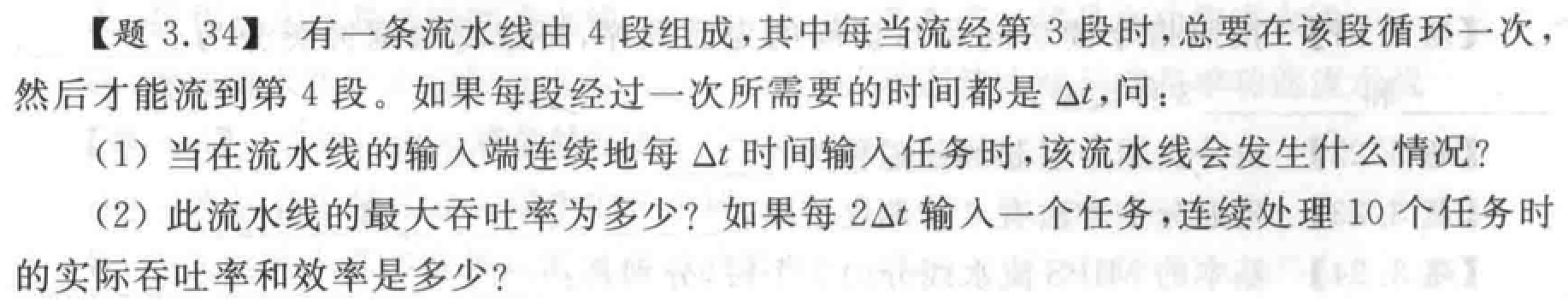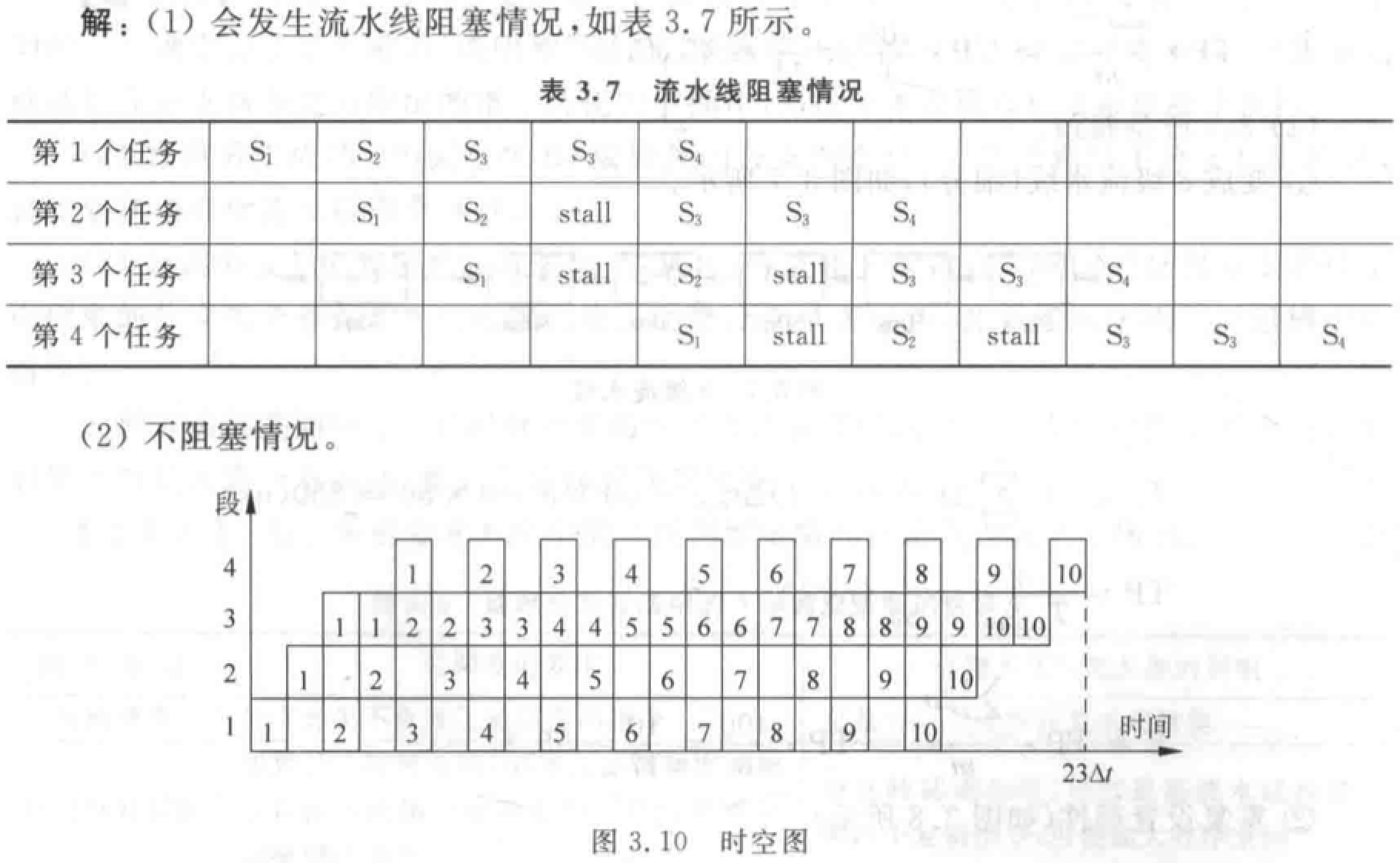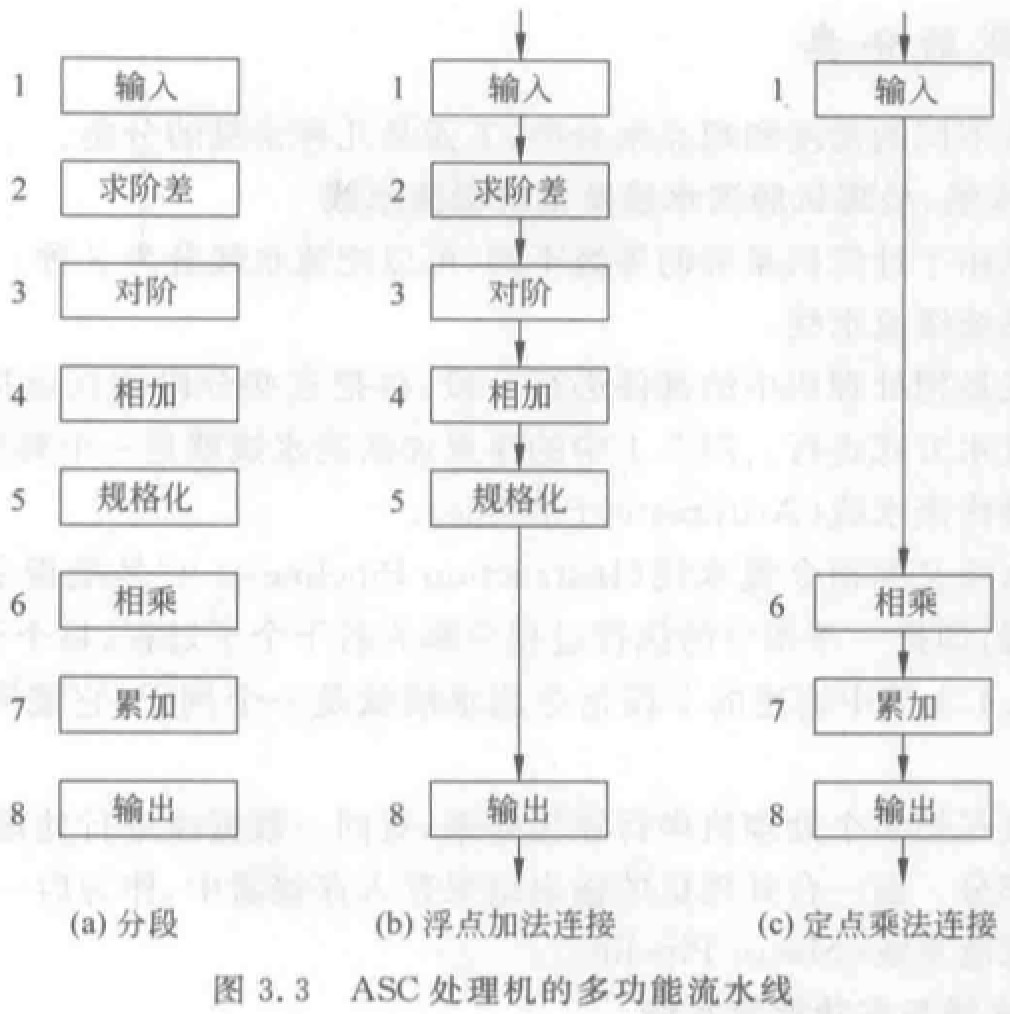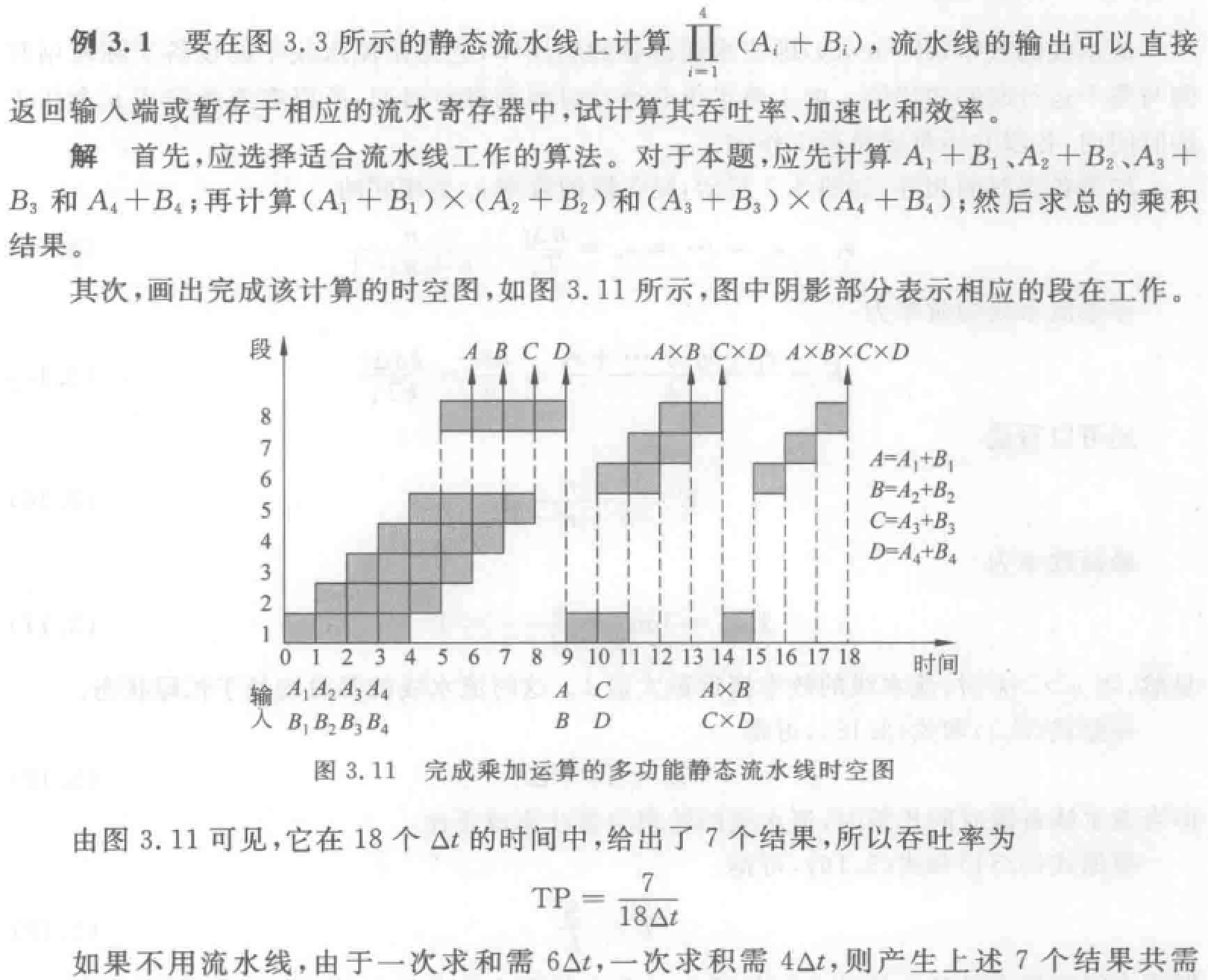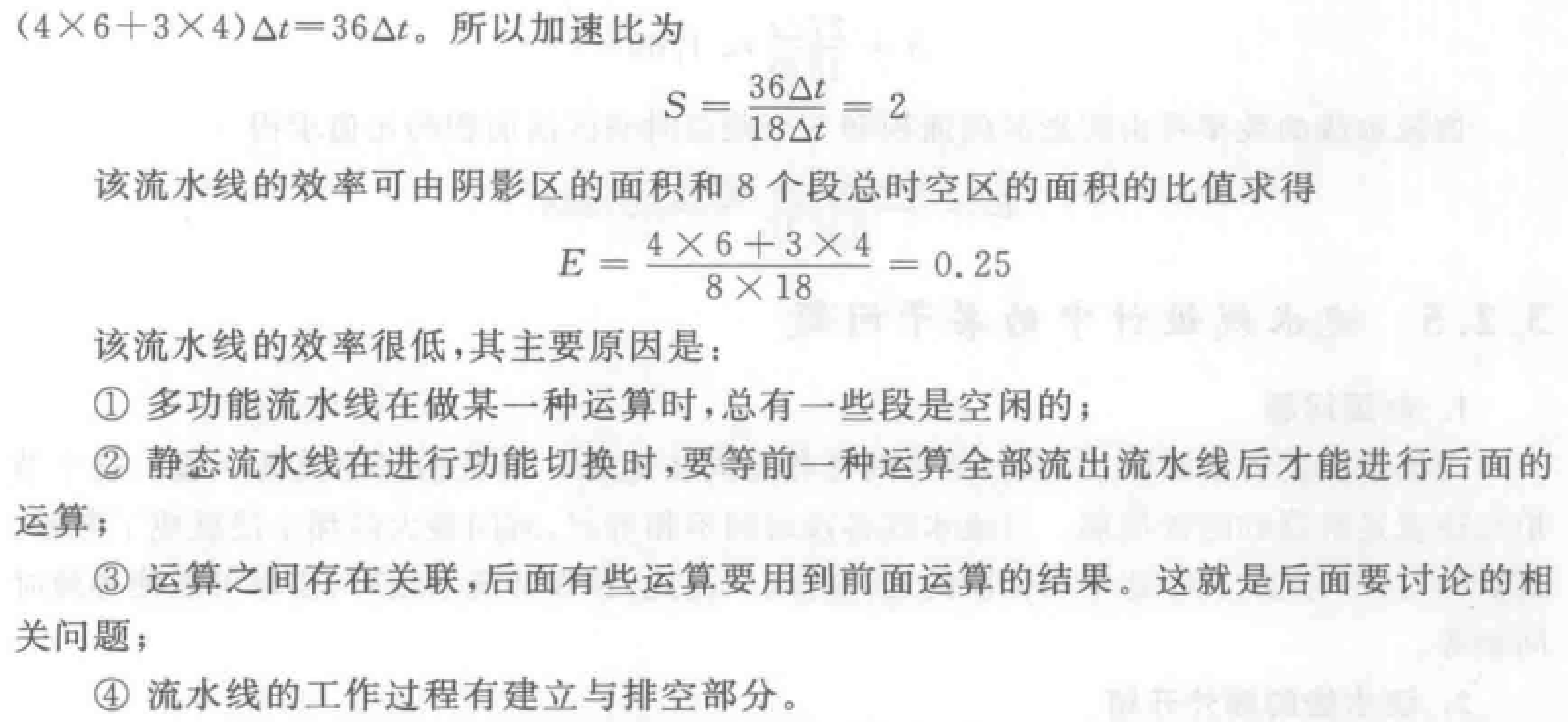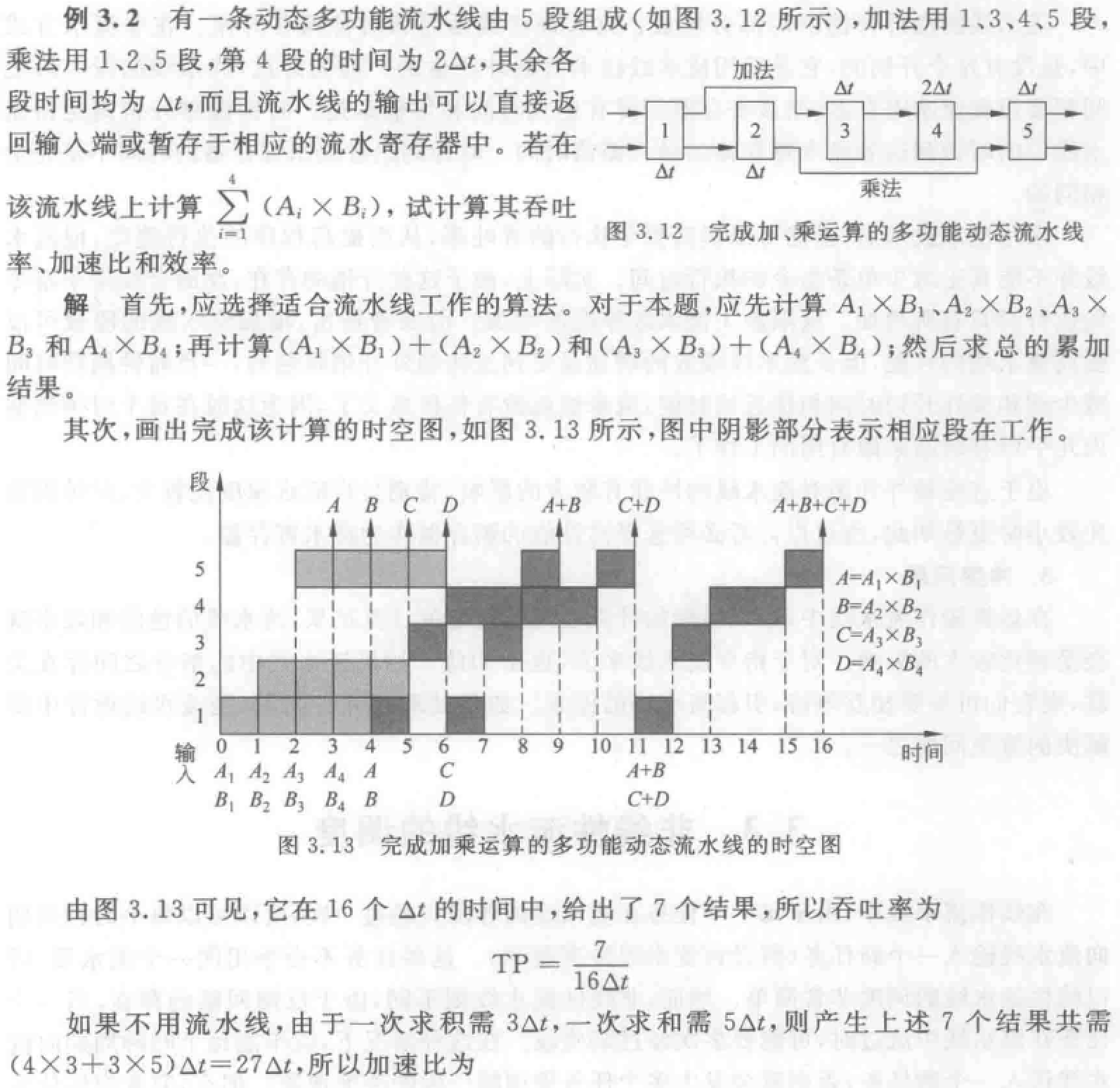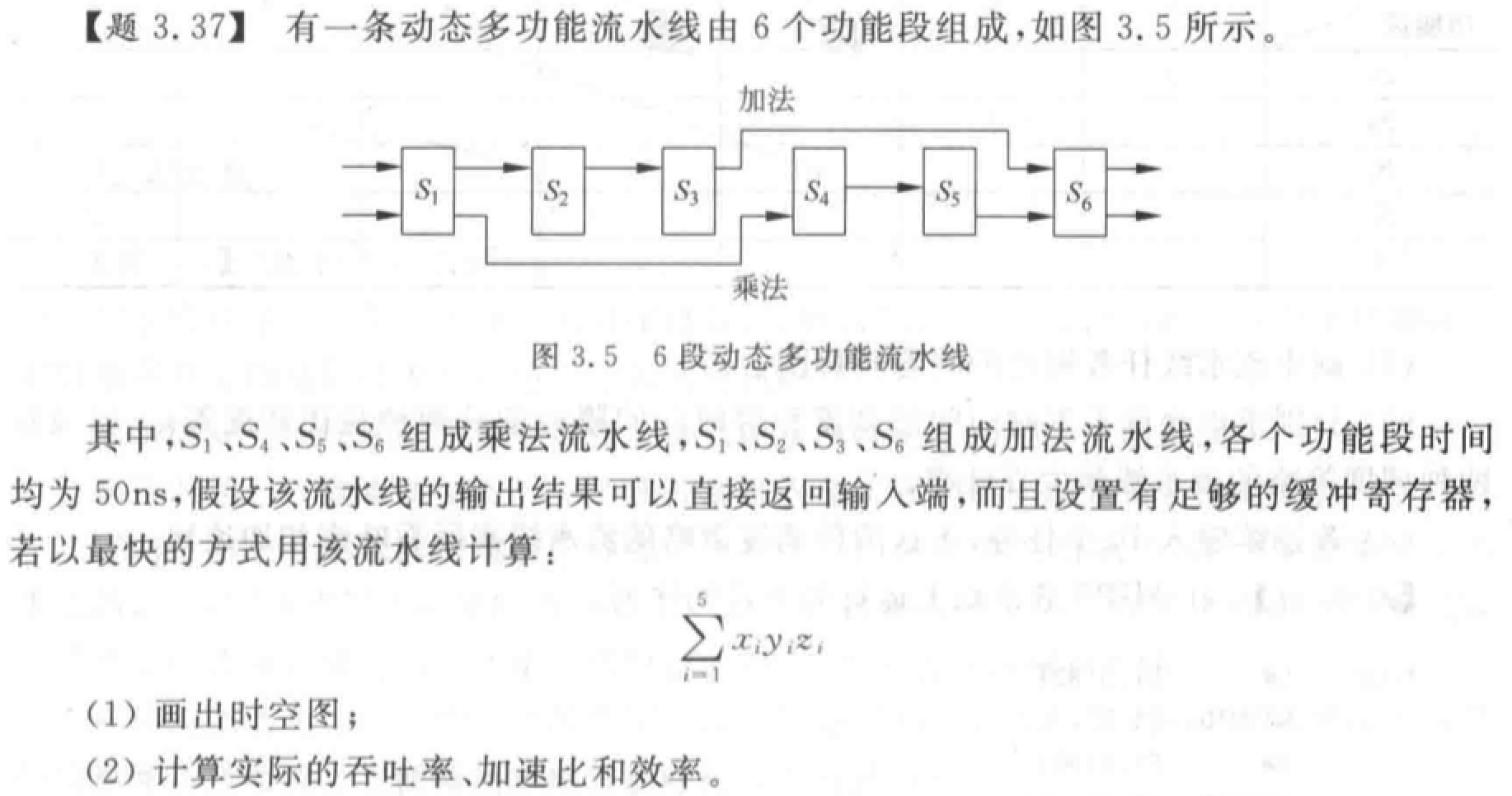## 单功能非线性流水线的最优调度*

1. 根据预约表写出禁止表 F。

2. 根据禁止表 F 写出冲突向量 $\mathbf{C_0}$

3. 根据出事冲突向量 $\mathbf{C_0}$ 画出状态转换图。

$\mathbf{C_k} = \mathbf{C_0}$，按下式计算新的冲突向量： $SHR^{(j)}(\mathbf{C_k})\vee \mathbf{C_0}$ 其中，$SHR^{(j)}$ 表示逻辑右移 $j$ 位。对于所有允许的时间间隔都按上述步骤求新的冲突向量，画出流水线状态转移图。

1. 根据状态转换图写出最优调度方案。由初始状态出发，任何一个闭合回路即为一种调度方案。列出所有方案，计算平均时间间隔，找出最小者。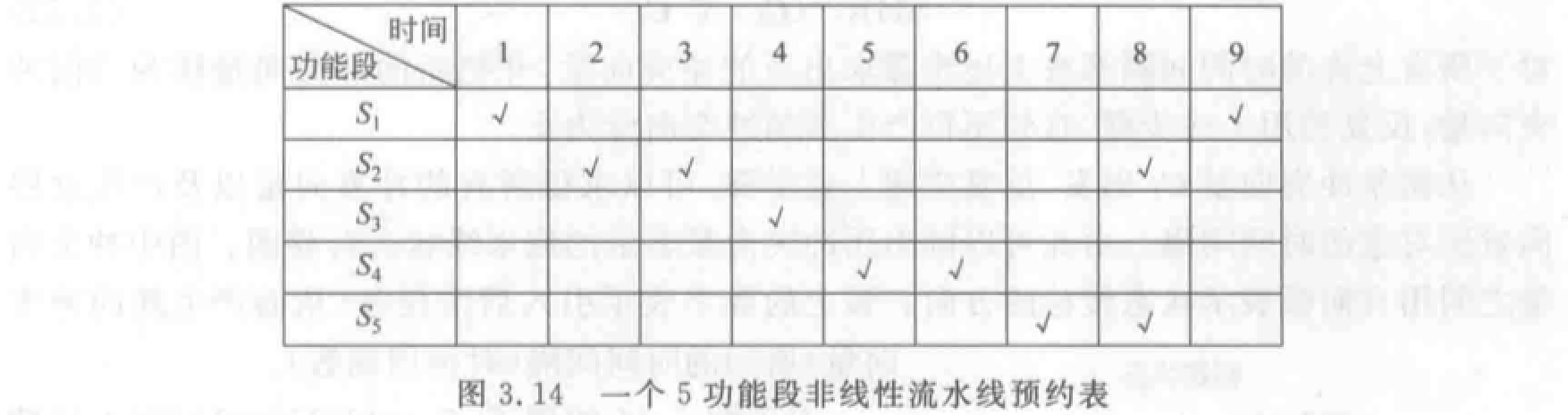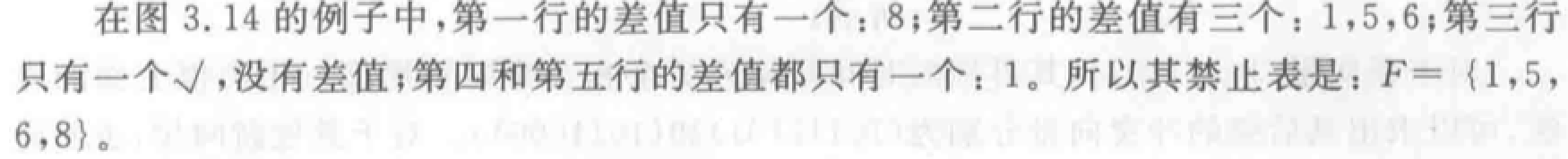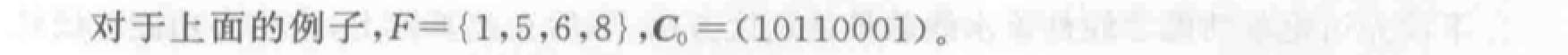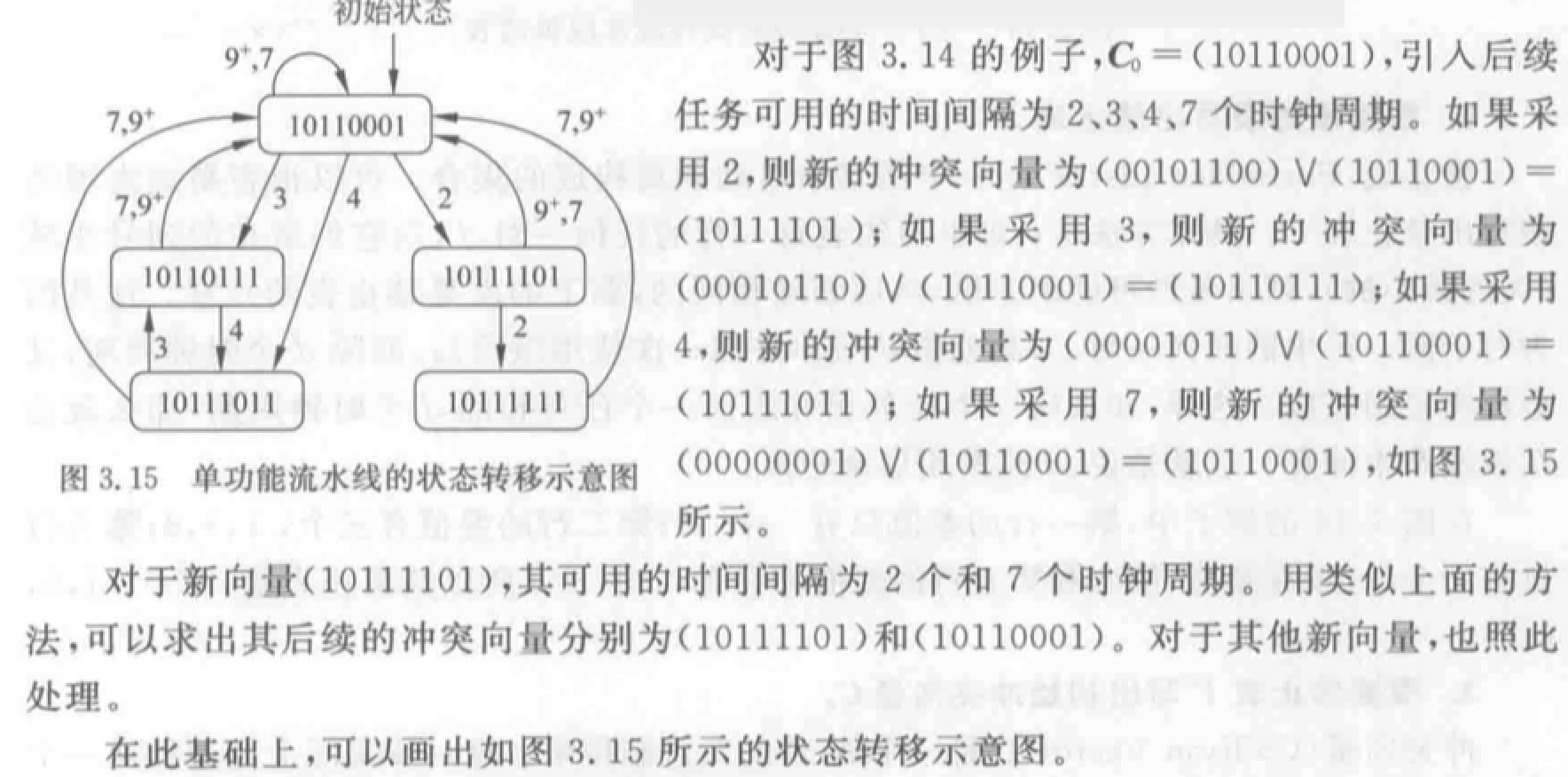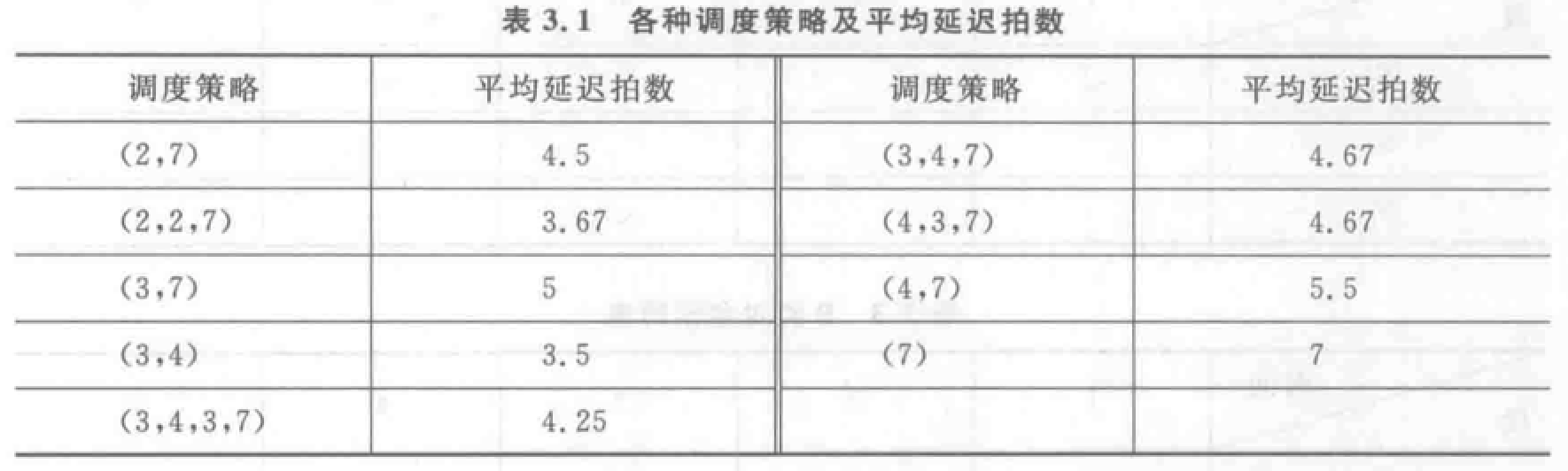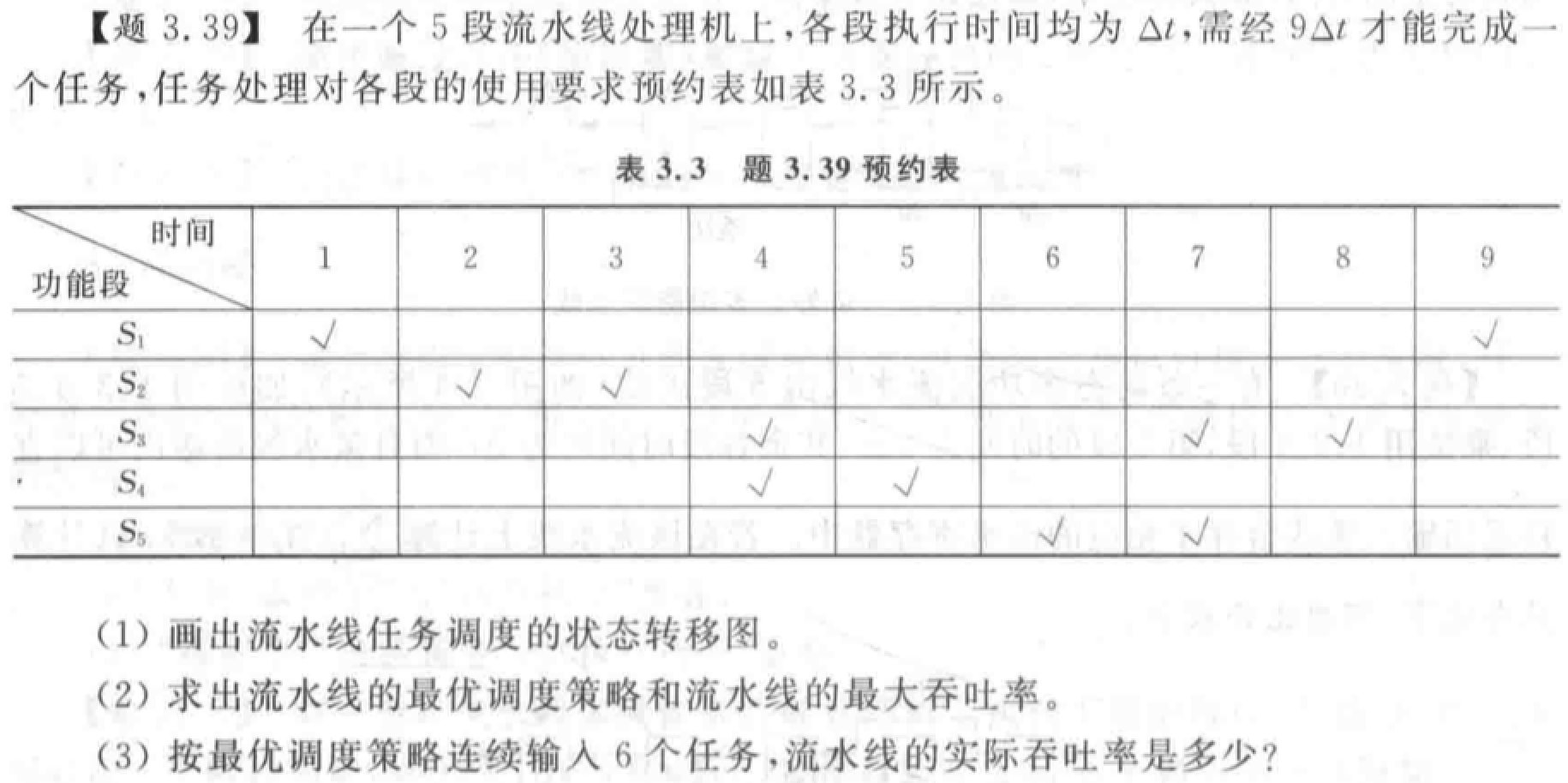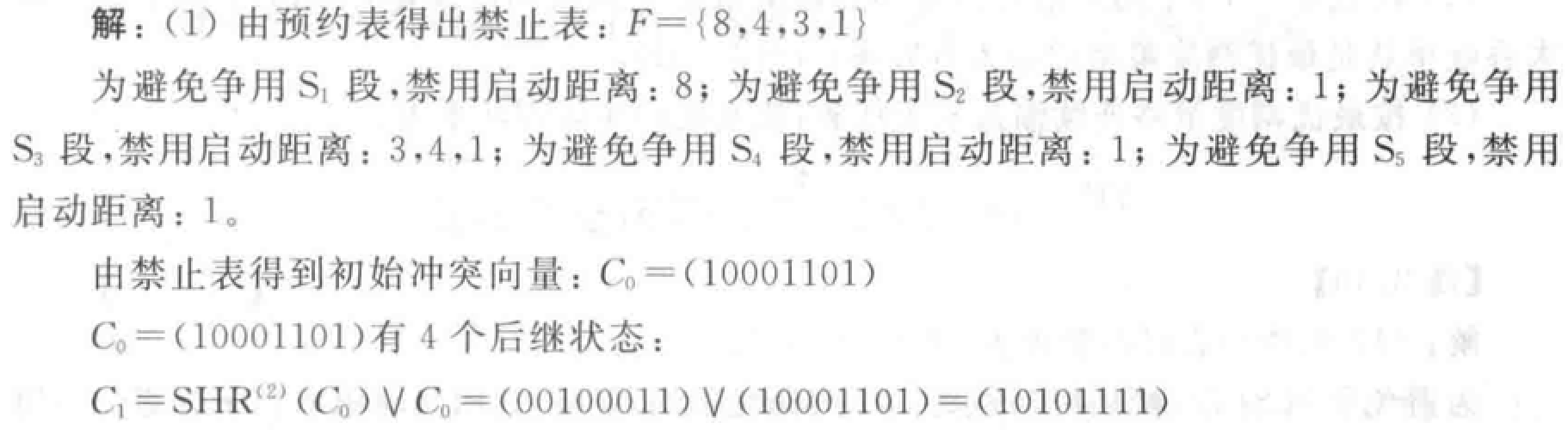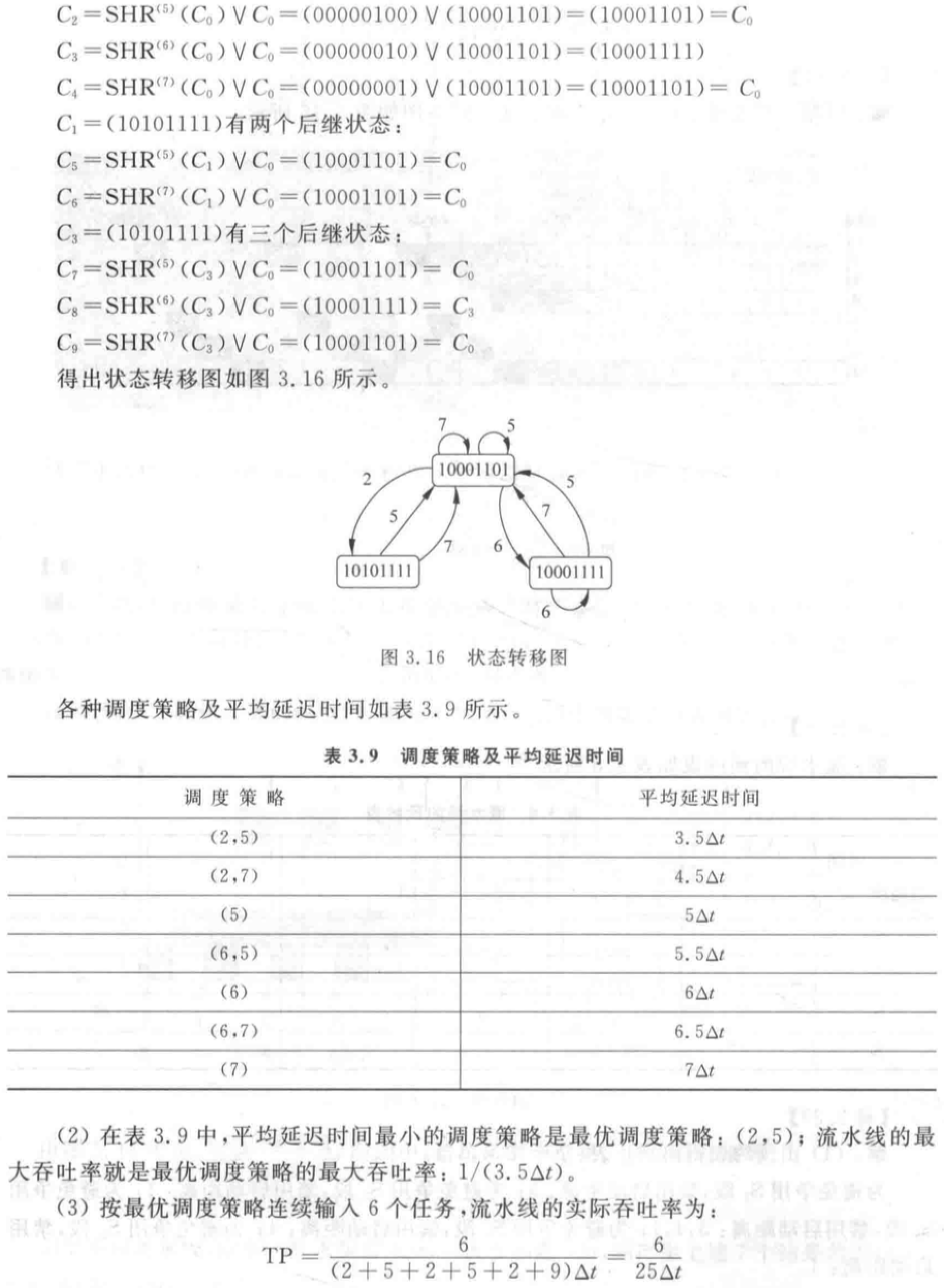## 流水线相关与冲突（五段流水线）

• IF：以程序计数器 PC 中的内容作为地址，从存储器中取出指令并放入指令寄存器 IR。PC 指向顺序的下一条指令。
• ID：指令译码，用 IR 中的寄存器地址去访问通用寄存器组，读出所需操作数。
• EX：
• loadstore 指令：ALU 把指定寄存器的内容与偏移量相加，形成访存有效地址。
• ALU 指令：ALU 对从通用寄存器组读出的数据进行运算。
• 分支指令：ALU 把偏移量与 PC 值相加，形成转移目标的地址。同时，判断分支是否成功。
• MEM：
• loadstore 指令：根据有效地址从存储器读出相应数据，或把指定数据写入有效地址指向的存储单元。
• 分支指令：如果分支成功，就把在前一个周期中计算好的转移目标地址送入 PC。分支指令执行完成。否则，不进行任何操作。
• ALU 指令此周期不进行操作。
• WB：把结果写入通用寄存器组。对于 ALU 指令，结果来自 ALU。对于 load 指令，结果来自存储器。

• 默认写操作在前半拍，读操作在后半拍。
• 如果是单周期延迟分支，则分支指令在 ID 段完成计算目标地址和判断分支是否成功。

• 相关：两条指令之间存在某种依赖关系，以至于他们可能无法在流水线中重叠执行，或只能部分重叠。

相关有三种类型：数据相关（真相关）、名相关、控制相关。

• 数据相关：下述条件之一成立，则称指令之间数据相关。

• 指令 a 使用指令 b 产生的结果。
• 指令 a 与指令 b 数据相关，而指令 b 与指令 c 数据相关。

第二个条件表明数据相关具有传递性。

• 名相关：名指指令访问的寄存器或存储器的名称。两条指令使用了相同的名，但并没有数据流动关系，则称为名相关。

• 反相关：指令 b 写的名与指令 a 读的名相同。反相关指令之间的执行顺序必须严格遵守，保证 b 读的值是正确的。
• 输出相关：指令 b 与指令 a 写的名相同。输出相关指令的执行顺序也必须严格遵守，保证最后的结果是指令 b 写进去的。

名相关的两条指令之间没有数据的传送，只是恰巧用了相同的名。可以通过换名技术（改变指令中操作数的名）消除名相关。对于寄存器操作数换名称为寄存器换名。寄存器换名既可以通过编译器静态实现，也可以硬件动态完成。

• 控制相关：分支指令和其它会改变 PC 值的指令引起的相关。需要根据分支指令的执行结果来确定后面该执行哪个分支上的指令。

• 流水线冲突：对于具体的流水线，由于相关的存在，指令流中的下一条指令不能在指定的时钟周期开始执行。有三种类型，结构冲突、数据冲突、控制冲突。约定：当一条指令被暂停时，在该指令之后流出的所有指令都要被暂停，而之前流出的指令仍继续进行。

• 结构冲突：某种指令组合因为硬件资源冲突而不能正常执行，称具有结构冲突。功能部件不是完全流水或硬件资源份数不够时发生。解决方法：插入暂停周期，或增加 Cache 等硬件资源。

• 数据冲突：相关的指令靠得足够近，他们在流水线中的重叠执行或重新排序会改变指令读/写操作数的顺序，使结果错误，谓数据冲突。

• 写后读冲突（RAW、WR）：对应真数据相关。
• 写后写冲突（WAW、WW）：对应输出相关。写后写冲突仅发生在“不止一个段可以进行写操作，或指令被重新排序”的流水线中。前述五段流水线不发生 WAW 冲突。
• 读后写冲突（WAR、RW）：对应反相关。读后写冲突仅发生在“有些指令的写结果操作被提前、有些指令的读操作被滞后，或指令被重新排序”的流水线中。前述五段流水线不发生 WAR 冲突。

使用定向技术（旁路技术）减少数据冲突引起的停顿：将计算结果从其产生的地方（ALU 出口）直接送到其他指令需要它的地方（ALU 的入口），可以避免停顿。

需要停顿的数据冲突（例如 LD 后接一个算术指令）：对于无法通过定向技术解决的数据冲突，需要设置一个“流水线互锁机制”的功能部件保证指令正确执行。其作用是检测和发现数据冲突，并使流水线停顿（stall）直至冲突消失。

依靠编译器解决数据冲突：在编译时让编译器重新组织指令顺序来消除冲突。称为指令调度流水线调度

• 控制冲突：分支指令和其它会改变 PC 值的指令引起的冲突。处理分支指令最简单的方法是“冻结”“排空”流水线，在 ID 段检测到分支指令时，立即暂停流水线输入，进行 EX、MEM，确定是否分支成功并计算出新的 PC 值，这样带来 3 个时钟周期的延迟。

为了减少分支延迟，可以采取：① 尽早判断出（或猜测）分支转移是否成功。② 尽早计算出分支目标地址。通过编译器减少分支延迟的方法：

• 预测分支失败：在检测到分支指令之后，沿分支失败的分支继续处理指令。当确定分支是失败时（预测分支失败成功），流水线正常流动。否则（预测分支失败失败），把在分支指令之后取出的指令转化为空操作，按分支目标地址重新取指执行。预测分支失败成功（分支失败）：0 延迟；预测分支失败失败（分支成功）：1 延迟。

• 预测分支成功：没用。除非已知分支目标地址。

• 延迟分支：把无论是否分支成功都必须执行的指令，紧接着分支指令执行（放入延迟槽），延迟槽中的指令替换了原本必须插入的暂停周期。绝大多数延迟槽仅容纳一条指令。

延迟槽指令的调度方法三种：

• 从前调度：从分支指令之前找一条指令插入延迟槽。被调度的指令必须与分支无关，适合任何情况。
• 从目标处调度：分支成功时起作用。分支成功概率高时采用。
• 从失败处调度：分支失败时起作用。不能从前调度时可用。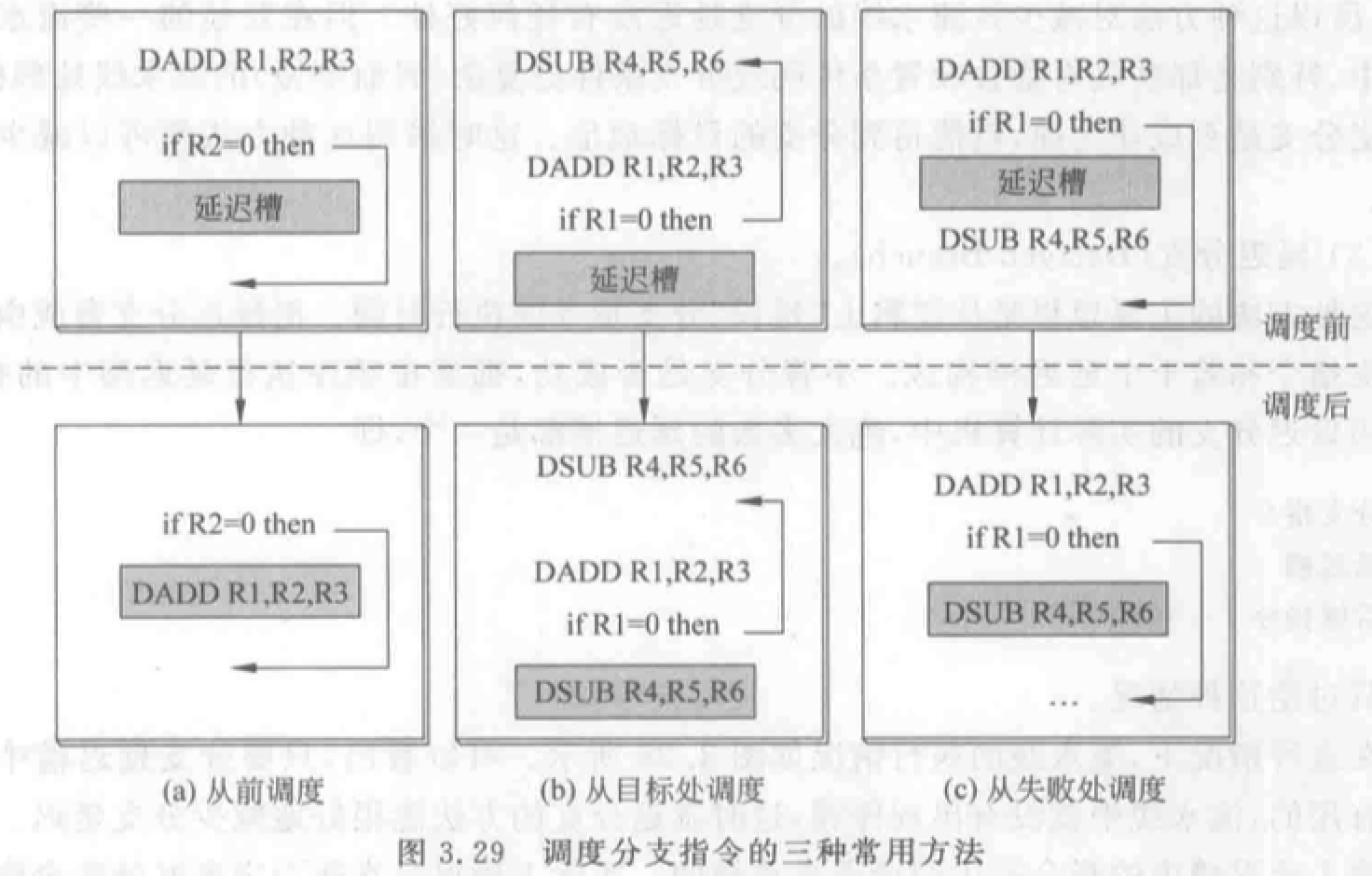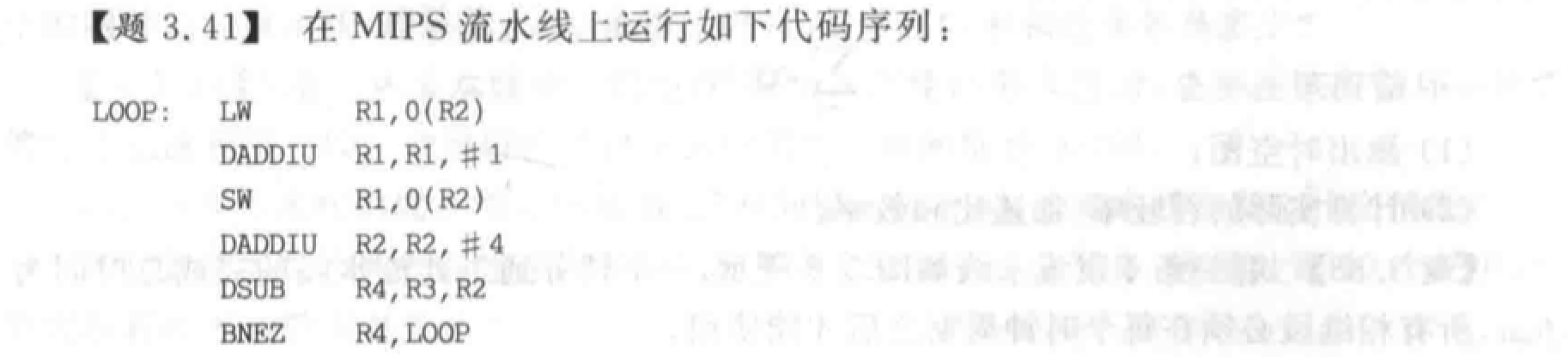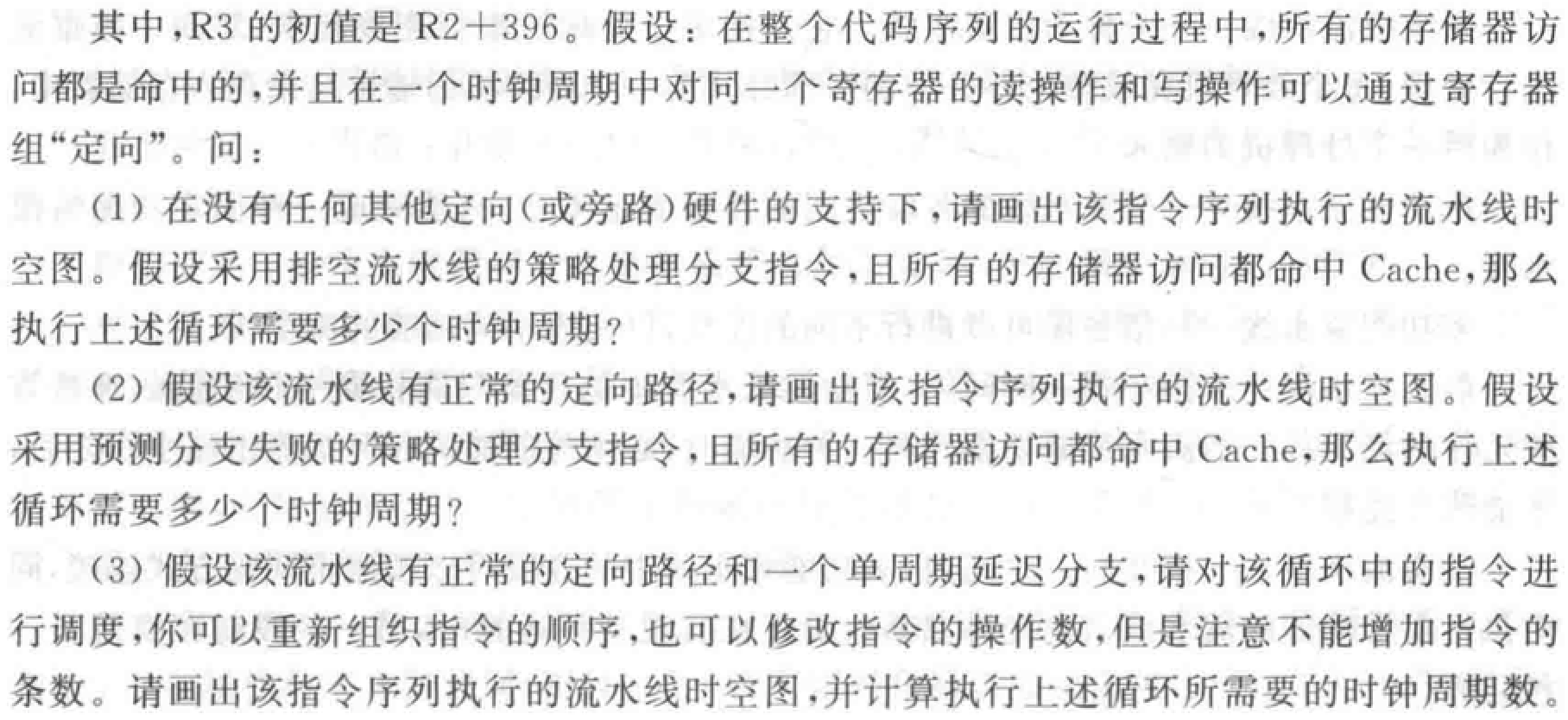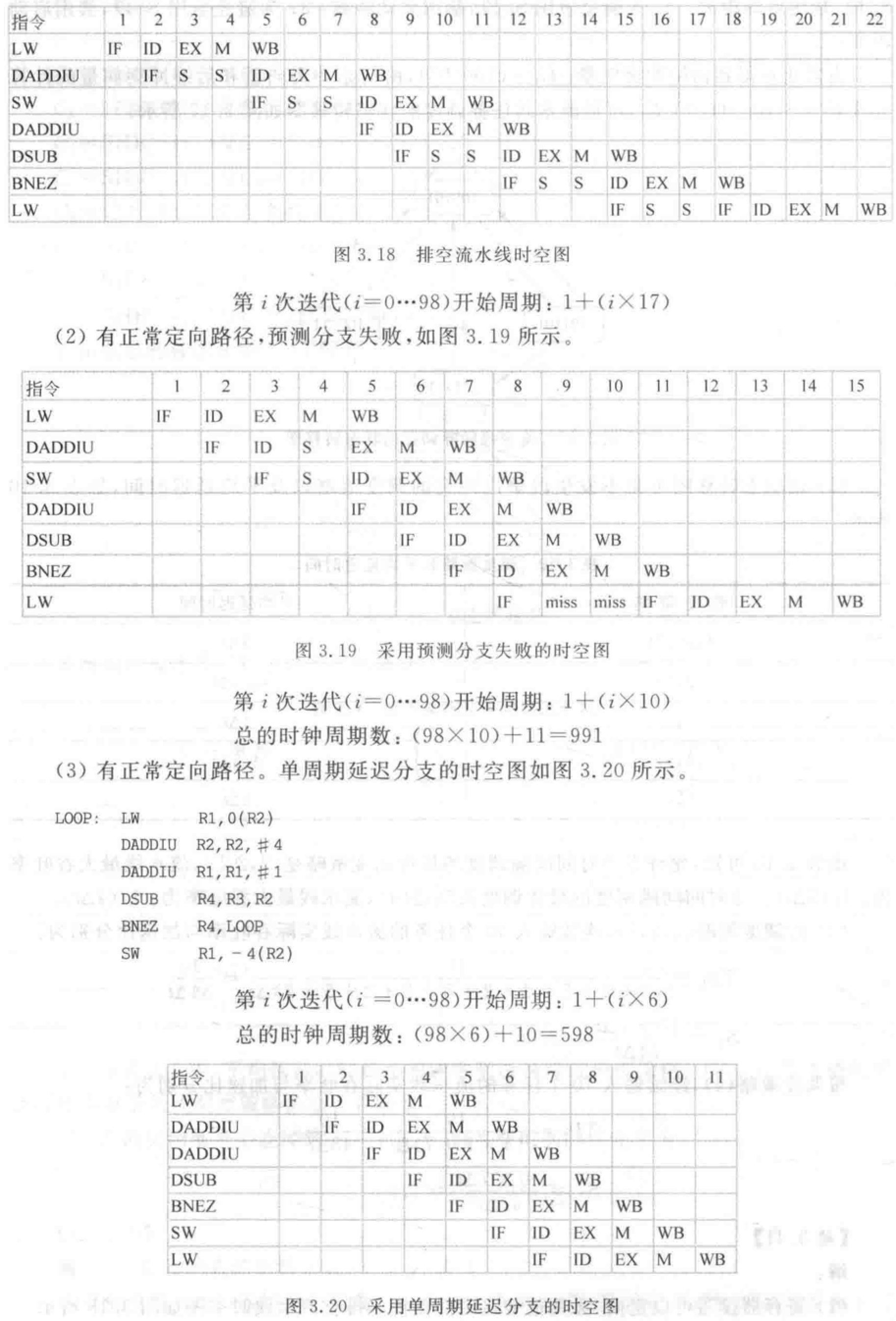1. 只有 WB 到 ID 的定向。例如 3、4 周期，停顿等待 WB 段写回寄存器，5 周期定向。 排空流水线，所以 15 周期 ID 段检测到分支指令就暂停下个指令的取指。15-17 周期，等待 MEM 写回 PC，18 周期才能取指。
2. 有定向之后，数据冲突不在 IF 停顿，在 ID 停顿。这道题是 3 周期延迟分支，所以预测分支失败失败会有 3 个周期的延迟。
3. 单周期延迟分支加上指令调度，就不会有延迟。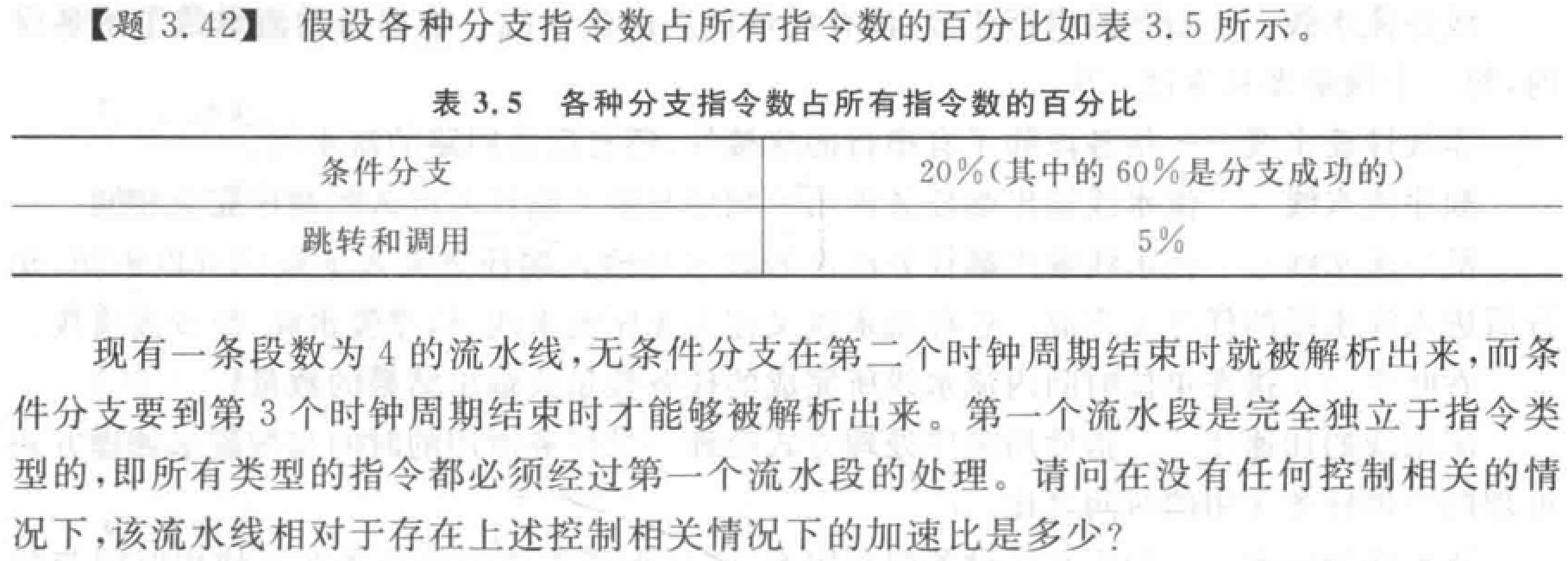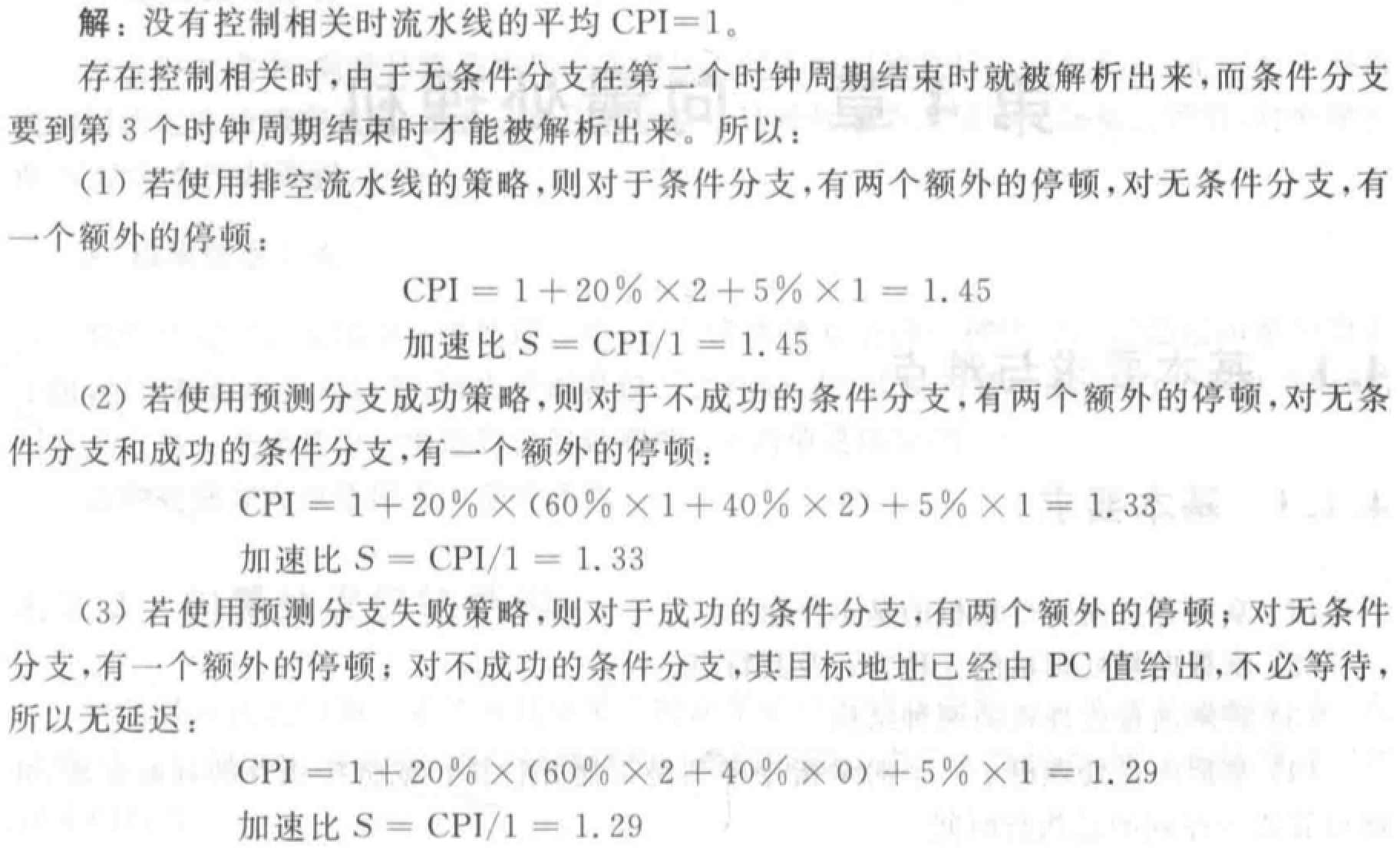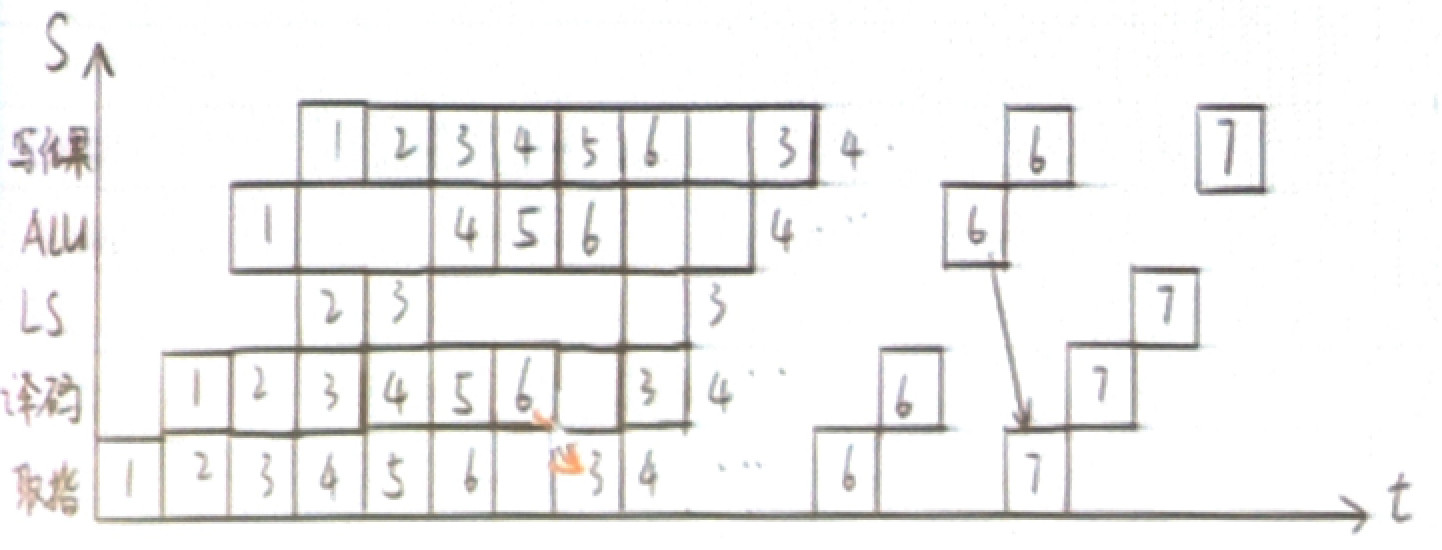（这道题题目里没说，但是看似是单周期延迟分支）

xqmmcqs

2022-06-06

2022-07-11